• python数据分析与挖掘实战一书的代码，属于python 大数据分析挖掘进阶版，数据挖掘实训平台的代码。 这本书你可以买翻版的，非常便宜，10元即可。学习起来亲。
• Python代码学习与挖掘实战，可以学习多一门语言和技术
• Python数据分析与挖掘 jupyter notebook
• 快速了解数据分析与挖掘技术 1.什么是数据分析与挖掘技术（概念） 所谓数据分析，即对已知的数据进行分析，然后提取出一些有价值的信息，比如统计出平均数、标准差等信息，数据分析的数据量有时可能不会太大，而...
快速了解数据分析与挖掘技术

1.什么是数据分析与挖掘技术（概念）

所谓数据分析，即对已知的数据进行分析，然后提取出一些有价值的信息，比如统计出平均数、标准差等信息，数据分析的数据量有时可能不会太大，而数据挖掘，是指对大量的数据进行分析与挖掘，得到一些未知的，有价值的信息等，比如从网站的用户或用户行为数据中挖掘出用户的潜在需求信息，从而对网站进行改善。

数据分析与数据挖掘密不可分，数据挖掘是数据分析的提升。

2.数据分析与挖掘技术能做什么事情（概念 ）

数据挖掘技术可以帮助我们更好的发现事务之间的规律，所以，我们可以利用数据挖掘技术实现数据规律的探索、比如发现窃电用户、发掘用户潜在需求、实现信息的个性化推送、发现疾病与症状设置疾病与药物之间的规律...等

3.数据挖掘的过程主要有（怎么实现）

定义目标
获取数据（常用手段有通过爬虫采集或者下载一些统计网站发布的数据，并没有严格的数据把控）
数据探索（对我们数据的初步的研究，发现数据的特征，就知道我们接下来怎么处理）
数据预处理（数据清洗【去掉脏数据】、数据集成【集中】、数据变换【规范化】、数据规约【精简】）
挖掘建模（分类算法、聚类算法、关联算法、预测算法）
模型评价与发布
2.数据分析与挖掘相关模块简介与安装

1.相关模块简介

numpy可以高效处理数据、提供数组支持、很多模块都依赖他，比如pandas、scipy、matplotlib都依赖他，所以这个模块是基础。
pandas在后续用的最多的一个模块，主要用于进行数据探索和数据分析
matplotlib 作图模块，解决可视化问题
scipy 主要进行数值计算，同时支持矩阵运算，并提供了恩多高等数据处理功能，比如积分、傅里叶变换、微分方程求解等。
statsmodels这个模块主要用于统计分析
Gensim这模块主要用于文本挖掘
skearn、keras前者机器学习，后者深度学习
2.相关模块的安装与技巧（建议这样安装）

1.numpy、mkl（下载安装numpy+mkl） 提取码：n5ac  （可以自行选择安装版本numpy+mkl）

2.pandas （网络安装）

3.matplotlib （网络安装）

4.scipy（下载安装，网盘下载建议先下载在安装）提取码：i383   网站下载

5.statsmodels  （网络安装）

6.Gensim（网络安装）

3.相关模块的基本使用

1. numpy（数组的运算效率要比列表的运算效率要高，底层运算是不一样的）

1. #创建一维数组

>>> import numpy
>>> x = numpy.array(['a','v','b'])  #  创建数组的格式
>>> x
array(['a', 'v', 'b'], dtype='<U1')    # 返回对应的元素，和对应的类型，取值按照下标取，如：x  返回  v

#创建二维数组

>>> x = numpy.array([[4,5,6,7],[8,9,7,6],[12,23,34,8]])

>>> x

array([[ 4,  5,  6,  7],
[ 8,  9,  7,  6],
[12, 23, 34,  8]])   #返回数据

2.排序  sort()     按照上面例子如：x.sort()

3.取最大值和最小值

按照上面例子 ：取最大值 ：x.max()

取最小值： x.min()

4.切片

按照上面例子：x[起始下标：最终下标+1]

2.pandas使用

import pandas as pda
'''
Series   index索引未指定按照0开始    行列pandas里面的一维数组
DataFrame  表格行和列格式  二维数组
'''
a = pda.Series([8.9, 2, 1]) #索引未指定
b = pda.Series([8,9, 2, 1],index=["one", "two", "three", "four"])#指定索引，index必须和数据相对应

#第一种方法
data = pda.DataFrame([[54, 1, 21, 24], [7, 58, 88, 99], [47, 85, 98, 72], [69, 58, 47, 25]]) #默认行列以0开始
data = pda.DataFrame([[54, 1, 21, 24], [7, 58, 88, 99], [47, 85, 98, 72], [69, 58, 47, 25]],columns=['one','two','three','four']) #修改列名，必须跟数据长度一样
#第二种方法，按照字典创建  (one，two，three）为列表名
data_dict =pda.DataFrame({
'one':4,         一列一行
'two':[6,2,3],   value值是列表为多行，
'three':list(str(982))
})   #其他行不满足时，自动补全，如：

》》》   one  two three
0    4    6     9
1    4    2     8
2    4    3     2

b.tail()       #尾部数据，默认后五行
b.tail(行数)   #自定义取尾部数据几行
data.sort_values(,ascending=False) #排序，参数【0】为列名，ascending=False  降序

展示统计情况：
data.describe() #把数据的统计信息给打印出来
返回：
one        two      three       four
count   4.000000   4.000000   4.000000   4.000000  # 元素个数的信息
mean   44.250000  50.500000  63.500000  55.000000  # 代表的平均数
std    26.474831  35.369478  35.911929  36.905284  # 展示标准差的意思
min     7.000000   1.000000  21.000000  24.000000  # 这一列最小值
25%    37.000000  43.750000  40.500000  24.750000  # 代表每一列，分位数
50%    50.500000  58.000000  67.500000  48.500000  #
75%    57.750000  64.750000  90.500000  78.750000  #
max    69.000000  85.000000  98.000000  99.000000  # 这一列最大值

#数据转置（将行变成列，将列变成行）
data.T  #转置行遍列

3.matplotlib基础 折线图/散点图 以及绘制子图

# 折线图、散点图  一般会有 plot模块绘制
import matplotlib.pylab as pyl
import numpy as npy

# 维数x轴和y轴必须一致，否者报错
x = [1, 2, 3, 4, 8]  # x轴
y = [5, 7, 2, 1, 5]  # y轴
pyl.plot(x, y)  # plot(x轴，y轴，展现形式)一般会有两个或者3个参数，第一个x轴数据，第二是y轴参数，第三是对应展示的形式(如：或者展示为散点图，设置展示颜色 )
pyl.show()  # 展示折线图，默认折线图
pyl.plot(x, y, 'o')  # 字母o是代表散点图
pyl.show()  # 折线图如果不注释，将会和下面的散点图重叠展示
pyl.plot(x, y, 'c')  # 默认折线图，换成青色
pyl.plot(x, y, 'oc')  # 修改成散点图，换成青色，是否是折线图的参数和颜色的参数叠加在一起
pyl.show()
# 颜色代表
'''
c-cyan--青色
r-red--红色
m-magente-品红
g-green-绿色
b-blue-蓝色
y-yellow-huangs
k-black-黑色
w-white-白色
'''
# 线条样式 常用线条
'''
-    直线
--   虚线
-.-. 形式(展示就是-.形式)
:     细小虚线
'''
# pyl.plot(x, y, '--')  # 折线图修改线条
# 点的样式
'''
s   方形
h   六角形
H   六角形(跟h有一点区别)
*   星形
x   x形
d   菱形
D   菱形(与d有点区别)
p   五角星
'''
pyl.plot(x, y, "*")  # 加上点的样式，默认是散点图
pyl.show()
# 设置标题以及x轴与y轴名称
pyl.plot(x, y)
x2 = [1, 3, 6, 8, 10, 12, 19]
y2 = [1, 6, 9, 10, 19, 23, 35]
pyl.plot(x2, y2)
pyl.title('show')  # 标题
pyl.xlabel('ages')  # x轴名称
pyl.ylabel('temp')  # y轴名称
pyl.xlim(0, 20)  # 设置x轴的范围
pyl.ylim(1, 40)  # 设置y轴的范围
pyl.show()  # 使用多图绘制，在show之前，多次使用plot

随机数的生成
# 前提导入
import numpy as npy

# data = npy.random.random_integers(1, 20, 10)  # 生成整型数值，第一参数，最小值，第二参数：最大数，第三个参数：随机数的个数
# 怎么生成正太分布的随机数，生成正太分布使用normal模块
data=npy.random.normal(5.0,2.0,10)  # 均数，西格玛(越小越陡峭，越大越平缓)，个数
print(data)

# 随机数网址 http://www.mamicode.com/info-detail-507676.html
# 直方图  一般会有hist模块  绘制
# 直方图一般统计某一段数据的次数
#正太分布随机
data = npy.random.normal(10.0, 1.0, 10000)
pyl.hist(data)
pyl.show()
#整型随机
data1=npy.random.random_integers(1,25,1000)
pyl.hist(data1)
pyl.show()

# 直方图的宽度以及上下限
sty = npy.arange(2, 19, 2) #x轴开始和结束值，第三参数是数据范围条件
pyl.hist(data1,sty,histtype='stepfilled')#第二个参数不叫，显示默认的，histtype='stepfilled'取消直方图轮廓
pyl.show()

# 绘制子图
pyl.subplot(2, 2, 4)  # 参数1代表行，参数2代表列，参数3代表当前区域
pyl.show()
# 绘制两行一列

pyl.subplot(2, 2, 1)  # 共两行第一行分两列第一列
x1 = [1, 2, 3, 4, 5]
y1 = [5, 3, 5, 23, 5]
pyl.plot(x1, y1)

pyl.subplot(2, 2, 2)  # 共两行第一行分两列第2列
x2 = [5, 2, 3, 8, 6]
y2 = [7, 9, 12, 12, 3]
pyl.plot(x2, y2)

pyl.subplot(2, 1, 2)  # 共两行第二行分一列第2行
x3=[5,6,7,10,19,20,29]
y3=[6,2,4,21,5,1,5]
pyl.plot(x3,y3)
pyl.show()


展开全文• Python数据分析与挖掘实战》的ppt 内容详细 讲解清楚
• Python数据分析与挖掘实战学习笔记-------------Chapter2 主要讲解了运用数据挖掘相关的几个扩展库进行数据分析数据的挖掘
• python数据分析与挖掘实战是一本python语言数据分析和挖掘指南，由张良均，王路，谭立云和苏剑林等十余个专家编著而成。本书以大家熟知的数据挖掘建模工具Python语言来展开，以解决某个应用的挖掘目标为前提，先介绍...
python数据分析与挖掘实战是一本python语言数据分析和挖掘指南，由张良均，王路，谭立云和苏剑林等十余个专家编著而成。本书以大家熟知的数据挖掘建模工具Python语言来展开，以解决某个应用的挖掘目标为前提，先介绍案例背景提出挖掘目标，再阐述分析方法与过程，最后完成模型构建，在介绍建模过程中穿插操作训练，把相关的知识点嵌入相应的操作过程中，使读者轻松理解并掌握相关的理论和知识点。python数据分析与挖掘实战从实践出发，结合大量数据挖掘工程案例及教学经验，以真实案例为主线，深入浅出地介绍数据挖掘建模过程中的有关任务：数据探索、数据预处理、分类与预测、聚类分析、时序预测、关联规则挖掘、智能推荐和偏差检测等，能够广泛应用于物联网、云计算、移动互联网等战略性新兴产业。内容介绍《python数据分析与挖掘实战》共15章，分两个部分：基础篇、实战篇。基础篇介绍了数据挖掘的基本原理，实战篇介绍了一个个真实案例，通过对案例深入浅出的剖析，使读者在不知不觉中通过案例实践获得数据挖掘项目经验，同时快速领悟看似难懂的数据挖掘理论。读者在阅读过程中，应充分利用随书配套的案例建模数据，借助相关的数据挖掘建模工具，通过上机实验，以快速理解相关知识与理论。基础篇（第1～5章），第1章的主要内容是数据挖掘概述；第2章对本书所用到的数据挖掘建模工具Python语言进行了简明扼要的说明；第3章、第4章、第5章对数据挖掘的建模过程，包括数据探索、数据预处理及挖掘建模的常用算法与原理进行了介绍。实战篇（第6～15章），重点对数据挖掘技术在电力、航空、医疗、互联网、生产制造以及公共服务等行业的应用进行了分析。在案例结构组织上，本书是按照先介绍案例背景与挖掘目标，再阐述分析方法与过程，最后完成模型构建的顺序进行的，在建模过程的关键环节，穿插程序实现代码。最后通过上机实践，加深读者对数据挖掘技术在案例应用中的理解。python数据分析与挖掘实战章节目录前言基础篇第1章数据挖掘基础1.1某知名连锁餐饮企业的困惑1.2从餐饮服务到数据挖掘1.3数据挖掘的基本任务1.4数据挖掘建模过程1.4.1定义挖掘目标1.4.2数据取样1.4.3数据探索1.4.4数据预处理1.4.5挖掘建模1.4.6模型评价1.5常用的数据挖掘建模工具1.6小结每2章Python数据分析简介2.1搭建Python开发平台2.1.1所要考虑的问题2.1.2基础平台的搭建2.2 Python使用入门2.2.1运行方式2.2.2基本命令2.2.3数据结构2.2.4库的导入与添加2.3 Python数据分析工具2.3.1 Numpy2.3.2 Scipy2.3.3 Matplotlib2.3.4 Pandas2.3.5 StatsModels'''''2.3.6 Scikit-Leam'''''2.3.7 Keras2.3.8 Gensim2.4配套资源使用设置2.5小结第3章数据探索3.1数据质量分析3.1.1缺失值分析3.1.2异常值分析3.1.3 -致性分析3.2数据特征分析3.2.1分布分析3.2.2对比分析3.2.3统计量分析?3.3Python主要数据探索函数3.3.1基本统计特征函数3.3.2拓展统计特征函数3.3.3统计作图函数3.4小结数据预处理4.1数据清洗4.1.1缺失值处理4.1.2异常值处理4.2数据集成4.2.1实体识别4.2.2冗余属性识别413数据变换4.3.1简单函数变换4.3.2规范化4.3.3连续属性离散化4.3.4属性构造4.3.5小波变换4.4数据规约4.4.1属性规约4.4.2数值规约4.5 Python主要数据预处理函数4.6小结第5章挖掘建模5.1分类与预测5.1.1实现过程5.1.2常用的分类与预测算法5.1.3回归分析5.1.4决策树5.1.5人工神经网络5.1.6分类与预测算法评价5.1.7 Python分类预测模型特点 -5.2聚类分析5.2.1 常用聚类分析算法5.2.2 K-Means聚类算法5.2.3聚类分析算法评价5.2.4 Python主要聚类分析算法5.3关联规则5.3.1 常用关联规则算法5.3.2 Apriori算法5.4时序模式5.4.1时间序列算法5.4.2时间序列的预处理5.4.3平稳时间序列分析5.4.4非平稳时间序列分析5.4.5 Python主要时序模式算法5.5离群点检测 1345.5.1离群点检测方法5.5.2基于模型的离群点检测方法5.5.3基于聚类的离群点检测方法5.6小结实战篇第6章电力窃漏电用户自动识别6.1背景与挖掘目标6.2分析方法与过程6.2.1数据抽取6.2.2数据探索分析6.2.3数据预处理6.2.4构建专家样本6.3上机实验6.4拓展思考6.5小结第7章航空公司客户价值分析7.1背景与挖掘目标7.2分析方法与过程7.2.1数据抽取7.2.2数据探索分析7.2.3数据预处理7.2.4模型构建7.3上机实验7.4拓展思考 一7.5小结第8章中医证型关联规则挖掘8.1背景与挖掘目标8.2分析方法与过程8.2.1数据获取8.2.2数据预处理8.2.3模型构建8.3上机实验8.4拓展思考8.5小结第9章基于水色图像的水质评价9.1背景与挖掘目标9.2分析方法与过程9.2.1数据预处理9.2.2模型构建9.2.3水质评价9.3上机实验9.4拓展思考9.5小结第10章家用电器用户行为分析与事件识别10.1背景与挖掘目标10.2分析方法与过程10.2.1数据抽取10.2.2数据探索分析10.2.3数据预处理10.2.4模型构建10.2.5模型检验10.3上机实验10.4拓展思考10.5小结第11章应用系统负载分析与磁盘容量预测11.1背景与挖掘目标11.2分析方法与过程11.2.1数据抽取11.2.2数据探索分析11.2.3数据预处理11.2.4模型构建11.3上机实验11.4拓展思考11.5小结第12章电子商务网站用户行为分析及服务推荐12.1背景与挖掘目标12.2分析方法与过程12.2.1数据抽取12.2.2数据探索分析12.2.3数据预处理12.2.4模型构建12.3上机实验12.4拓展思考12.5小结第13章财政收入影响因素分析及13.1预测模型13.2背景与挖掘目标分析方法与过程13.2.1灰色预测与神经网络的组合模型13.2.2数据探索分析13.2.3模型构建13.3上机实验13.4拓展思考13.5小结 一第14章 基于基站定位数据的商圈分析 一14.1背景与挖掘目标14.2分析方法与过程14.2.1数据抽取14.2.2数据探索分析14.2.3数据预处理14.2.4模型构建14.3上机实验14.4拓展思考14.5小结第15章电商产品评论数据情感分析15.1背景与挖掘目标15.2分析方法与过程15.2.1评论数据采集15.2.2评论预处理15.2.3文本评论分词15.2.4模型构建15.3.上机实验15.4拓展思考15.5小结参考文献使用说明1、下载并解压，得出pdf文件2、如果打不开本文件，请务必下载pdf阅读器3、安装后，在打开解压得出的pdf文件4、双击进行阅读
展开全文• Python数据分析与挖掘实战第三章 数据探索3.1 数据质量分析3.1.1 缺失值分析3.1.2 异常值分析3.2 数据特征分析3.2.1 统计量分析3.2.2 贡献度分析3.2.2 相关性分析 第三章 数据探索 3.1 数据质量分析 3.1.1 缺失值...
Python数据分析与挖掘实战第三章 数据探索3.1 数据质量分析3.1.1 缺失值分析3.1.2 异常值分析3.2 数据特征分析3.2.1 统计量分析3.2.2 贡献度分析3.2.3 相关性分析3.2.4 统计特征函数3.2.5 绘图函数第四章 数据预处理4.1 数据清洗4.1.1 缺失值处理4.2 数据变换4.1.1 数据归一化4.1.2 数据离散化(聚类)4.3 属性改造4.4 数据规约4.5 常用预处理函数第五章 挖掘建模
第三章 数据探索
3.1 数据质量分析
3.1.1 缺失值分析
缺失值的处理分为三种情况:

删除存在缺失值的记录;
对可能的数据进行插值:拉格朗日插值,牛顿插值法:

3.1.2 异常值分析
首先可以先使用describe()函数查看数据的基本情况:
import pandas as pd
# 餐饮数据
catering_sale = './data/catering_sale.xls'
# 读取数据，指定日期列为索引列
data.describe()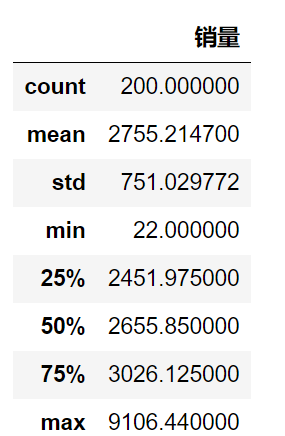检测异常值的方法可以使用箱型图:
import pandas as pd
catering_sale = './data/catering_sale.xls'
import matplotlib.pyplot as plt
# 用来正常显示中文标签
# plt.rcParams['dont.sans-serif'] = ['SimHei']
plt.rcParams['font.sans-serif'] = ['SimHei']    # 用来正常显示中文标签
# # 用来正常显示负号
plt.rcParams['axes.unicode_minus'] = False
plt.figure()
'''
画箱型图，这里画箱型图有两种方法:
1. 一种是直接调用DataFrame的boxplot()；
2. 另一种是调用Series或者DataFrame的plot()方法,并用kind参数指定箱型图(box);
'''
p = data.boxplot(return_type='dict')
# 'flies'即为异常值的标签
x = p['fliers'].get_xdata()
y = p['fliers'].get_ydata()
y.sort()
for i in range(len(x)):
if i > 0:
plt.annotate(y[i],xy = (x[i],y[i]), xytext = (x[i]+0.05-0.8/(y[i]-y[i-1]),y[i]))
else:
plt.annotate(y[i],xy = (x[i],y[i]),xytext = (x[i]+0.08,y[i]))
plt.show()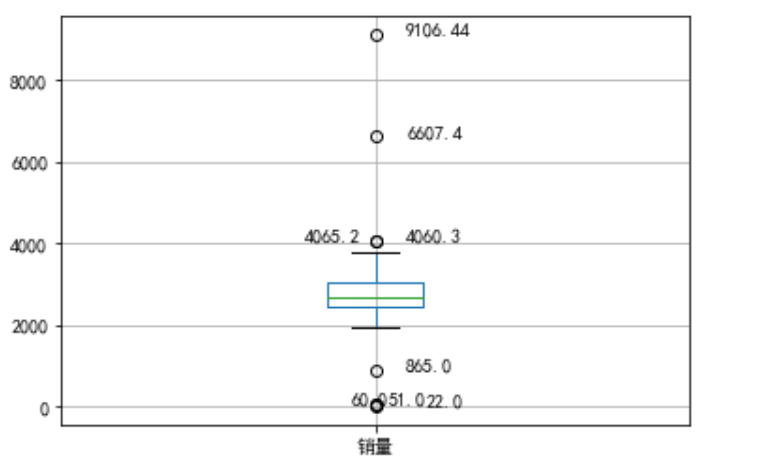上下的两个标线表示的是上界和下界(四分位点),超过上下界的值就是异常,但是那其中几个散点离上下界比较近,所以可以把865,4060.3,4065.2归为正常值,将22,51,60,6607.4,9106.44归为异常值;
3.2 数据特征分析
对于定量数据可以通过绘制频率分布表,绘制频率分布直方图,茎叶图的方式进行直观的分析;
对于定性分类的数据,可以使用饼图和条形图的方式来查看显示分布情况;
3.2.1 统计量分析
极差反映了最大值和最小值的分布情况;
标准差用来度量数据偏离均值的程度;
变异系数度量标准差相对于均值的离中趋势;
四分位数间距表示上下四分位数之差,越大表示变异程度越大;
# 餐饮销量数据统计量分析
import pandas as pd
catering_sale = './data/catering_sale.xls'
# 过滤异常数据
data = data[(data[u'销量'] > 400)&(data[u'销量'] < 5000)]
statistics = data.describe()
# 极差
statistics.loc['range'] = statistics.loc['max'] - statistics.loc['min']
# 变异系数
statistics.loc['var'] = statistics.loc['std'] / statistics.loc['mean']
# 四分位数间距
statistics.loc['dis'] = statistics.loc['75%'] - statistics.loc['25%']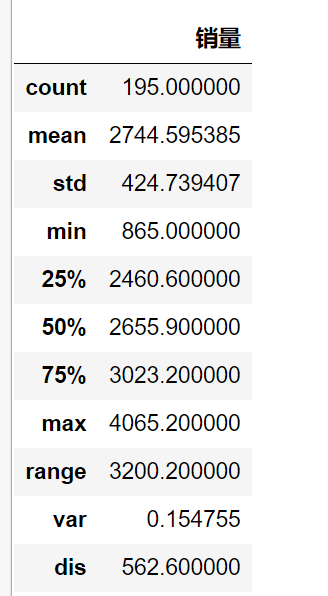3.2.2 贡献度分析
# 菜品盈利帕累托图代码
import pandas as pd
# 初始化参数
dish_profit = './data/catering_dish_profit.xls'
data = data[u'盈利'].copy()
# 表示按降序排列
data.sort_values(ascending=False)
# 导入图像库
import matplotlib.pyplot as plt
plt.rcParams['font.sans-serif'] = ['SimHei']    # 用来正常显示中文标签
plt.rcParams['axes.unicode_minus'] = False

plt.figure()
# 显示直方图
data.plot(kind='bar')
plt.ylabel(u'盈利(元)')
p = 1.0 * data.cumsum()/data.sum()
p.plot(color = 'r',secondary_y=True,style='-o',linewidth=2)
# 添加注释，即85%处的标记，这里包括了指定箭头样式
plt.annotate(format(p,'.4%'),xy=(6,p),xytext=(6*0.9,p*0.9),
plt.ylabel(u'盈利')
plt.show()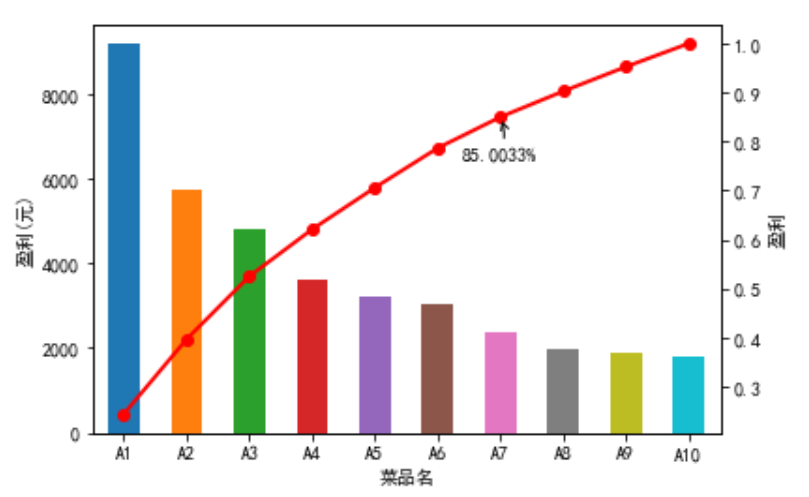3.2.3 相关性分析
import pandas as pd
# 餐饮数据，含有其他属性
catering_sale = './data/catering_sale_all.xls'
# 读取数据，指定'日期'列为索引列
# 相关系数矩阵,即给出了任意两款菜式之间的相关系数
data.corr()
# 只显示"百合酱蒸风爪"与其他菜式的相关系数
data.corr()[u'百合酱蒸凤爪']
# 计算两者的相关系数
data[u'百合酱蒸凤爪'].corr(data[u'翡翠蒸香茜饺'])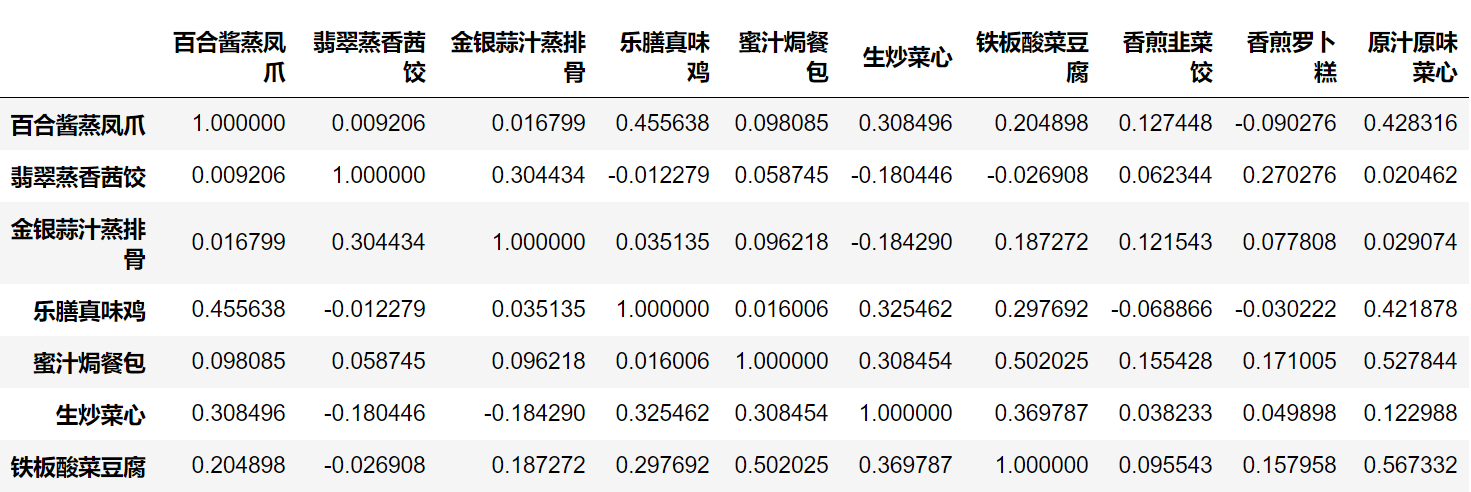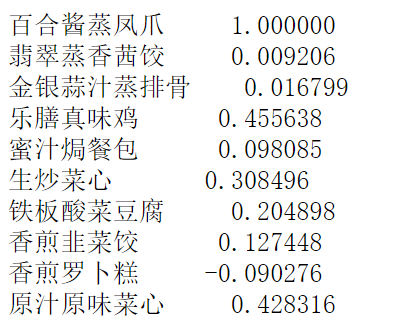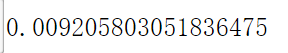计算两个列向量的相关系数:
# 计算两个列的相关系数
# 生成样本D,一行为1-7，一行为2-8
D = pd.DataFrame([range(1,8),range(2,9)])
# 提取第一行
S1 = D.loc
# 提取第二行
S2 = D.loc
# 计算S1，S2的相关系数:有person(皮尔逊系数),kendall(肯德尔系数),spearman(斯皮尔曼系数)
S1.corr(S2,method='pearson')

计算协方差矩阵:
# 计算协方差矩阵
import numpy as np
D = pd.DataFrame(np.random.randn(6,5)) # 产生6*5的随机矩阵
D.cov() #计算协方差矩阵
D.cov(D)  # 计算第一列和第二列的协方差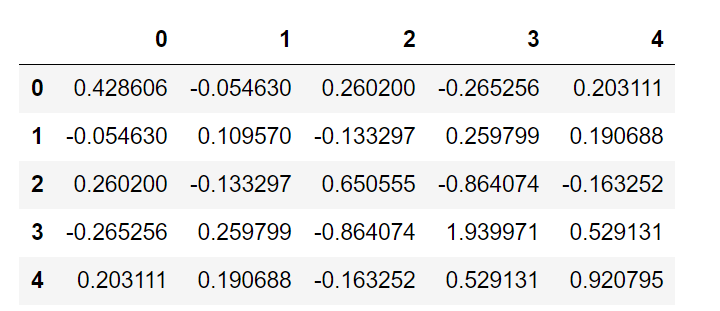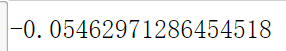计算 6 * 5 随机矩阵的偏度(三阶矩)/峰度(四阶矩)
# 计算 6 * 5 随机矩阵的偏度(三阶矩)/峰度(四阶矩)
import pandas as pd
D = pd.DataFrame(np.random.randn(6,5)) # 产生6*5的随机矩阵
# 偏度:通过对偏度系数的测量，我们能够判定数据分布的不对称程度以及方向
D.skew()
# 峰度:是研究数据分布陡峭或平滑的统计量，通过对峰度系数的测量，我们能够判定数据分布相对于正态分布而言是更陡峭还是平缓。
D.kurt()

3.2.4 统计特征函数
使用cumsum来获取某一列的前n项和:
# 使用cumsum来获取某一列的前n项和
D = pd.Series(range(0,20)) # 构造Series，内容为0-19共20个整数
D.cumsum(0) # 给出前n项和
# 问题：当其大于列数时，表示什么意思呢？

3.2.5 绘图函数
直线:
import matplotlib.pyplot as plt # 导入作图库
plt.rcParams['font.sans-serif'] = ['SimHei'] # 用来正常显示中文标签
plt.rcParams['axes.unicode_minus'] = False # 用来正常显示负号
plt.figure(figsize = (7,5)) # 创建图像区域，指定比例
import numpy as np
#设置起点,终点和步长
x = np.linspace(0, 2*np.pi, 50)  # x坐标输入
y = np.sin(x) # 计算对应x的正弦值
plt.plot(x, y, 'bp--') # 控制图形格式为蓝色带星虚线，显示正弦曲线
plt.show()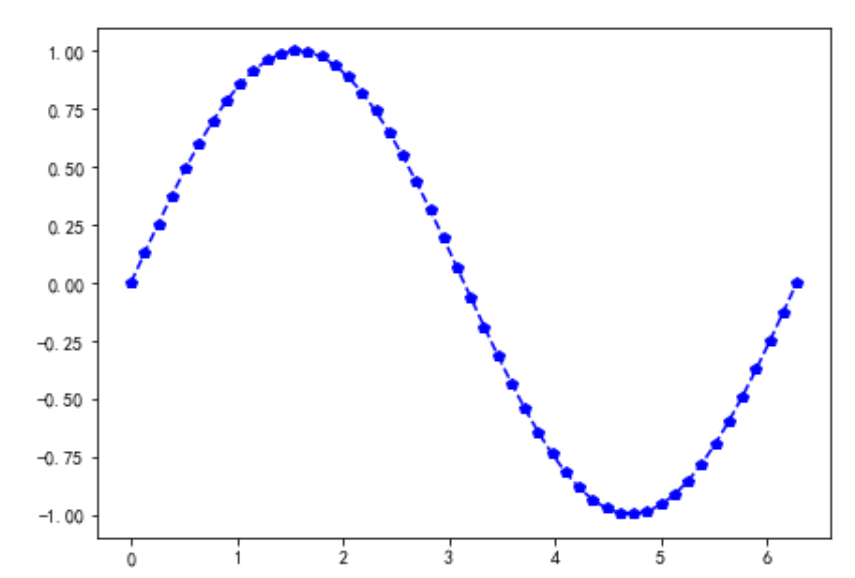饼图:
import matplotlib.pyplot as plt
labels = 'Frogs','Hogs','Dogs','Logs' # 定义标签
sizes = [15, 30, 45, 10] #每一块的比例
colors = ['yellowgreen', 'gold', 'lightskyblue', 'lightcoral'] #每一块的颜色
explode = (0,0.09,0,0) # 突出显示，这里仅仅突出第二块
plt.pie(sizes,explode=explode, labels=labels, colors=colors, autopct='%1.1f%%',
plt.axis('equal') #显示为圆(避免比例压缩为椭圆)
plt.show()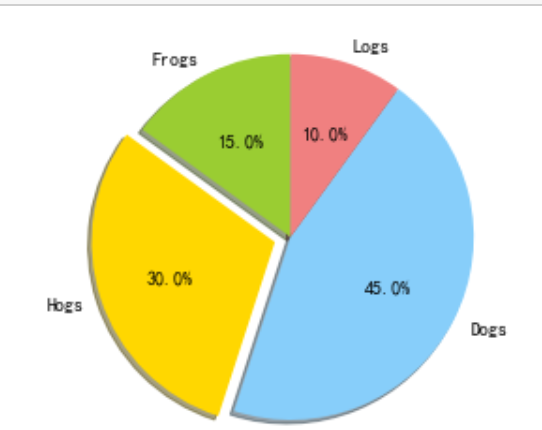直方图:
import matplotlib.pyplot as plt
import numpy as np
x = np.random.randn(1000) # 1000个服从正态分布的随机数
plt.hist(x, 10) # 分成10组进行绘制直方图
plt.show()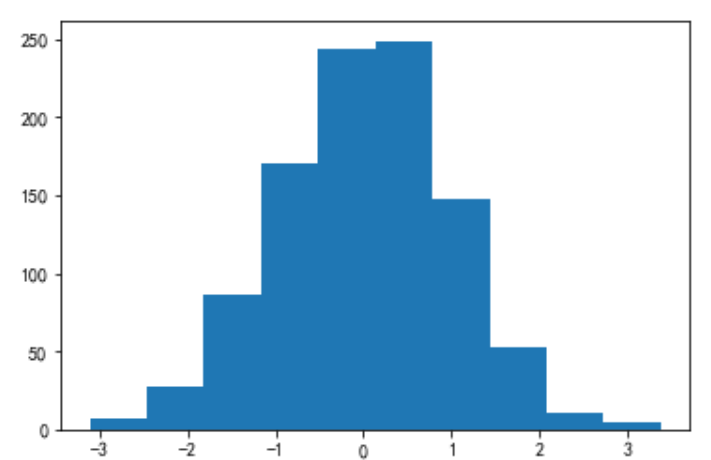箱型图
'''
绘制箱型图的两种方法:
1. 直接调用DataFrame的boxplot()方法；
2. 调用Series或者DataFrame的plot()方法,并用kind参数指定箱型图(box);
'''
import matplotlib.pyplot as plt
import numpy as np
import pandas as pd
x = np.random.randn(1000) # 1000个服从正态分布的随机数
D = pd.DataFrame([x,x+1]).T # 构造两列的DataFrame
D.plot(kind = 'box') # 调用Series内置的作图方法画图，用kind参数指定箱型图box
plt.show()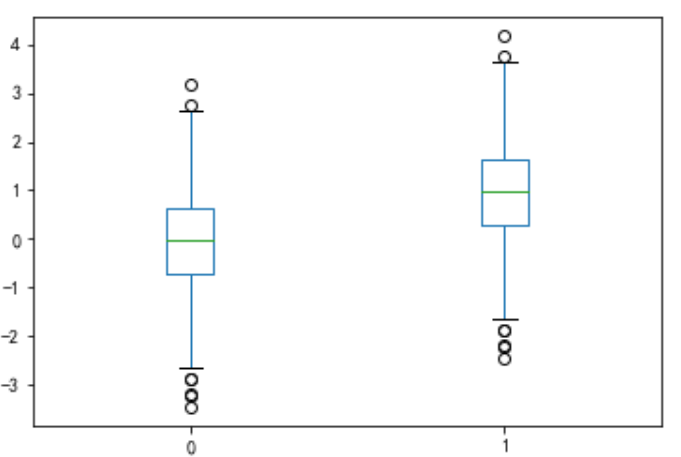折线图:
import matplotlib.pyplot as plt
plt.rcParams['font.sans-serif'] = ['SimHei'] # 用来正常显示中文标签
plt.rcParams['axes.unicode_minus'] = False # 用来正常显示负号

import numpy as np
import pandas as pd
# np.arrange()返回一个ndarray而不是一个列表
x = pd.Series(np.exp(np.arange(20))) # 原始数据
# 对图像左上方做好标记
x.plot(label = u'原始数据图',legend = True)
plt.show()
x.plot(logy = True, label = u'对数数据图',legend = True)
plt.show()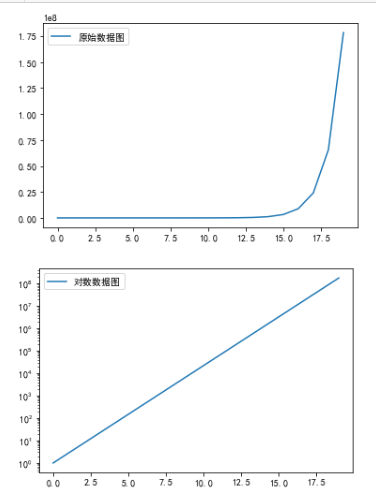import matplotlib.pyplot as plt
plt.rcParams['font.sans-serif'] = ['SimHei'] # 用来正常显示中文标签
plt.rcParams['axes.unicode_minus'] = False # 用来正常显示负号

import numpy as np
import pandas as pd
error = np.random.randn(10) # 定义误差列
y = pd.Series(np.sin(np.arange(10))) #均值数据列
y.plot(yerr = error) #绘制误差图
plt.show()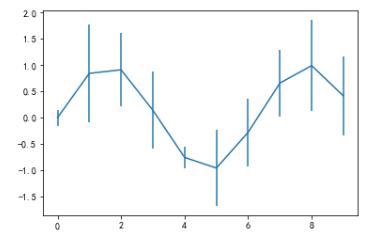第四章 数据预处理
4.1 数据清洗
4.1.1 缺失值处理
缺失值处理一般采用:均值/中位数/众数插补,使用固定值,最近邻值插补,回归法,插值法
拉格朗日插值:
# 用拉格朗日法进行插补
import pandas as pd
from scipy.interpolate import lagrange # 导入拉格朗日函数
import xlwt
inputfile = './data/catering_sale.xls' # 销售数据路径
outputfile = './sales.xls' # 输出数据路径
data[u'销量'][(data[u'销量'] < 400) | (data[u'销量'] > 5000)] = None # 过滤异常值，将其变为空值

# 自定义列向量插值函数
# s为列向量，n为被插值的位置，k为取前后的数据个数，默认为5
def ployinterp_column(s, n, k=5):
y = s[list(range(n-k,n)) + list(range(n+1, n+1+k))] # 取数
y = y[y.notnull()] # 剔除空值
return lagrange(y.index, list(y))(n) # 插值并返回插值结果

# 逐个元素判断是否需要插值
for i in data.columns:
for j in range(len(data)):
if (data[i].isnull())[j]: #如果为空即插值
data[i][j] = ployinterp_column(data[i],j)

data.to_excel(outputfile) # 输出结果, 写入文件

4.2 数据变换
4.1.1 数据归一化
# 数据规范化
import pandas as pd
import numpy as np
datafile = './data/normalization_data.xls' #参数初始化
(data - data.min())/(data.max() - data.min()) # 最小-最大规范化
(data - data.mean())/data.std() # 零-均值规范化
data/10**np.ceil(np.log10(data.abs().max())) # 小数定标规范化

4.1.2 数据离散化(聚类)
等宽法,等频法,基于聚类分析的方法;
def cluster_plot(d, k):  # 自定义作图函数来显示聚类结果
import matplotlib.pyplot as plt
plt.rcParams['font.sans-serif'] = ['SimHei'] # 用来正常显示中文标签
plt.rcParams['axes.unicode_minus'] = False # 用来正常显示负号
plt.figure(figsize = (8, 3))
for j in range(0, k):
plt.plot(data[d==j], [j for i in d[d==j]], 'o')
plt.ylim(-0.5, k-0.5)
return plt

# 数据离散化:根据某列的数值来进行分组
import pandas as pd
datafile = './data/discretization_data.xls' #参数初始化
data = data[u'肝气郁结证型系数'].copy()
k = 4
# 1. 等宽离散化,各个类别依次命名为0,1,2,3
d1 = pd.cut(data, k, labels= range(k))
# 2. 等频率离散化
w = [1.0*i/k for i in range(k+1)]
w = data.describe(percentiles = w)[4:4+k+1] # 使用describe函数自动计算分位数
w = w * (1-1e-10)
d2 = pd.cut(data, w, labels = range(k))

# 3. 基于聚类分析的方法
from sklearn.cluster import KMeans # 引入KMeans
kmodel = KMeans(n_clusters = k, n_jobs = 4) # 建立模型,n_jobs是并行数,一般等于CPU数较好
kmodel.fit(data.values.reshape((len(data), 1)))
c = pd.DataFrame(kmodel.cluster_centers_).sort_values(by=0) # 输出聚类中心,并且排序(默认随机排序)

# # python3中没有rolling_mean的方法,需要将其改为rolling.mean
w = c.rolling(2).mean().iloc[1:]
# w
# .mean(2).iloc[1:] # 相邻两项求中点,作为边界点
w =  + list(w) + [data.max()] # 把首末边界点加上
d3 = pd.cut(data, w, labels = range(k))

cluster_plot(d1, k).show()
cluster_plot(d2, k).show()
cluster_plot(d3, k).show()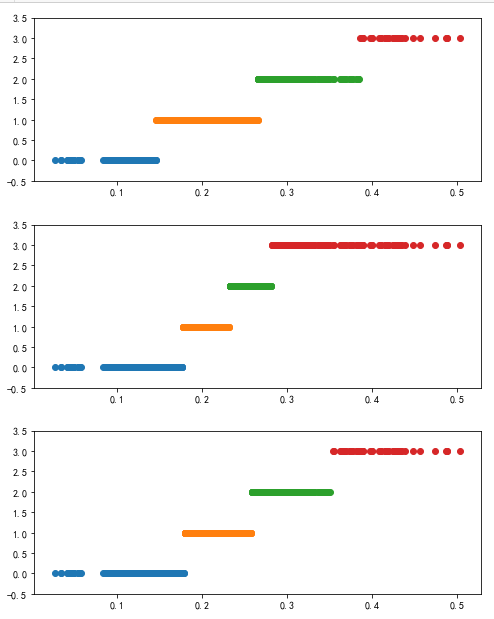4.3 属性改造
# 线损率属性改造
import pandas as pd
# 参数初始化
inputfile = './data/electricity_data.xls' #供入供出电量数据
outputfile = './electricity_data.xls' # 属性改造后的电量数据
data[u'线损率'] = (data[u'供入电量'] - data[u'供出电量'])/data[u'供入电量']
data.to_excel(outputfile, index = False) # 保存结果

4.4 数据规约
# 主成分分析降维代码
inputfile = './data/principal_component.xls'
outputfile = './dimention_reducted.xls' # 降维后的数据
from sklearn.decomposition import PCA
pca = PCA()
pca.fit(data)
pca.components_ # 返回模型的各个特征向量
pca.explained_variance_ratio_ # 返回各个成分各自的方差百分比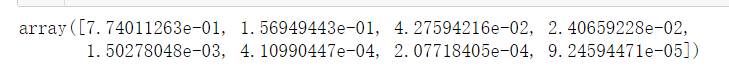# 使用pca的结果
pca = PCA(3)
pca.fit(data)
low_d = pca.transform(data) # 用它来降低维度
pd.DataFrame(low_d).to_excel(outputfile) # 保存结果
pca.inverse_transform(low_d) # 必要时可以用inverse_transform()函数来复原数据

4.5 常用预处理函数
D = pd.Series([1,1,2,3,5])
# 进行数据去重的两种方法
D.unique()
np.unique(D)

第五章 挖掘建模


展开全文数据分析 数据挖掘
• python数据分析与挖掘实战》读书笔记写的第3章到第5章的代码 记录我的学习之旅，每份文档倾心倾力，带我成我大牛，回头观望满脸笑意，望大家多多给予意见，有问题或错误，请联系 我将及时改正；借鉴文章标明出处，...
• Python数据分析与挖掘实战-----第2章 Python数据分析简介Python数据分析与挖掘实战-----第2章 Python数据分析简介搭建Python开发平台Python使用入门Python数据分析工具配套资源使用设置 Python数据分析与挖掘实战---...
Python数据分析与挖掘实战-----第2章 Python数据分析简介Python数据分析与挖掘实战-----第2章 Python数据分析简介搭建Python开发平台Python使用入门Python数据分析工具配套资源使用设置
Python数据分析与挖掘实战-----第2章 Python数据分析简介
搭建Python开发平台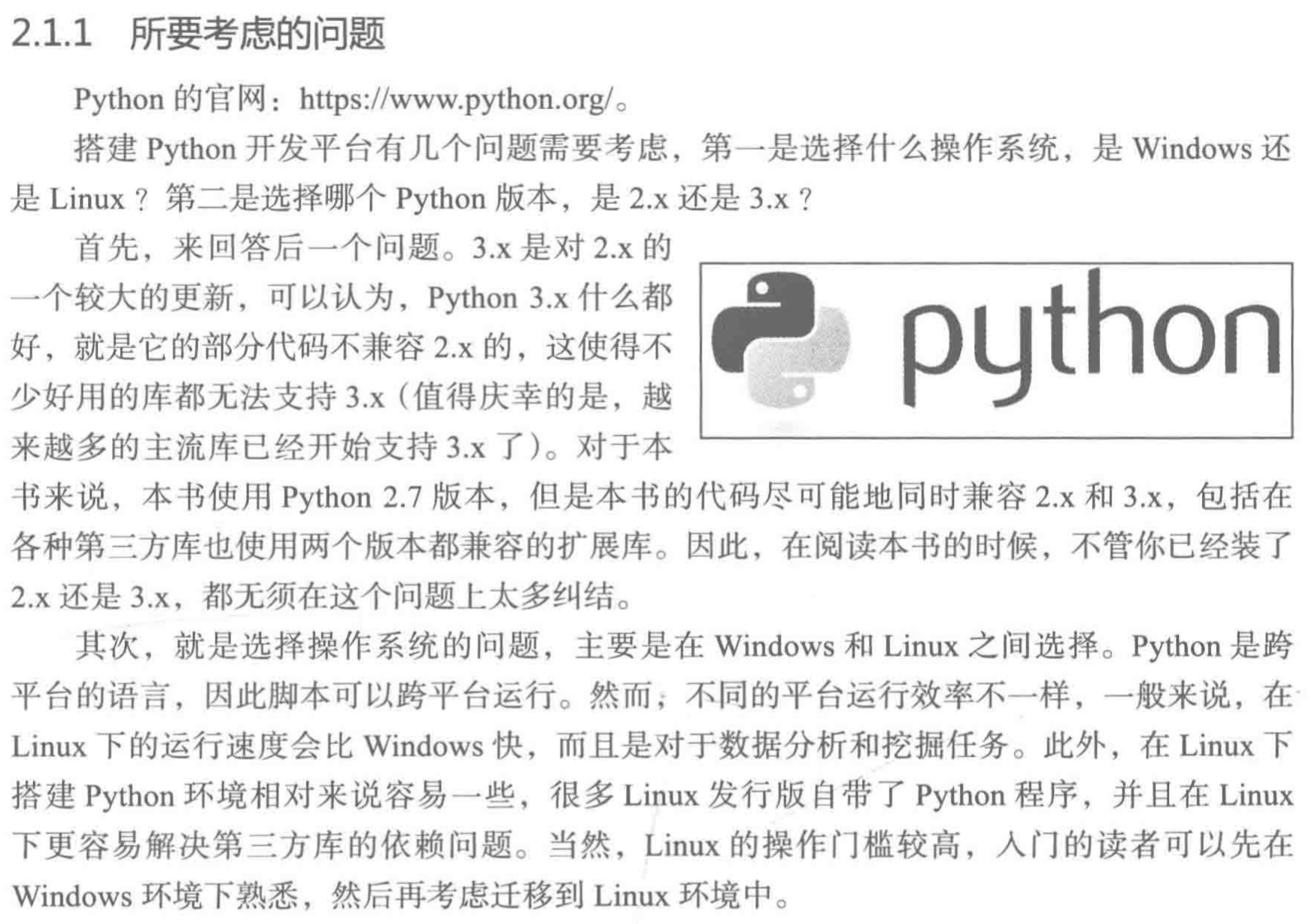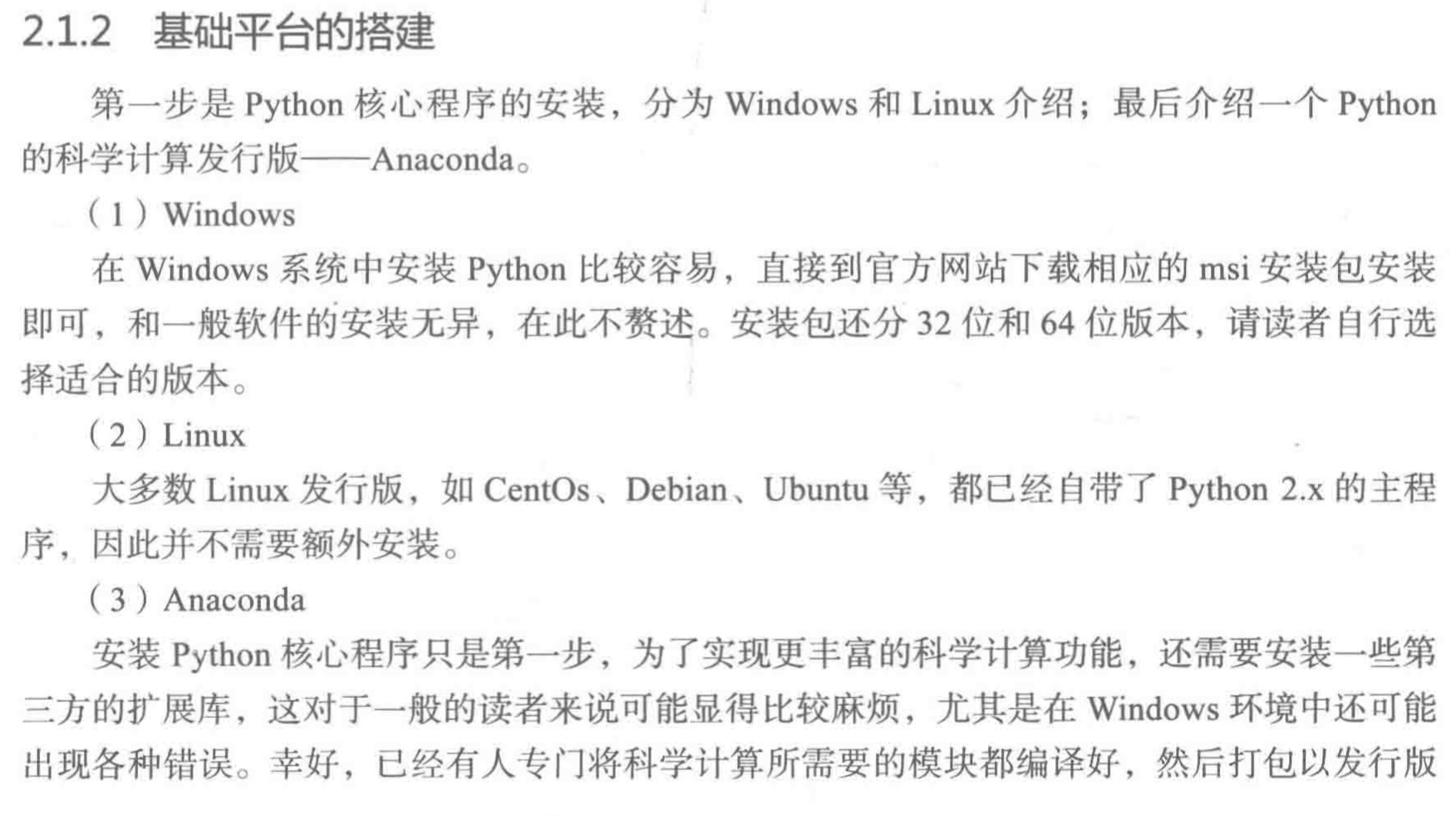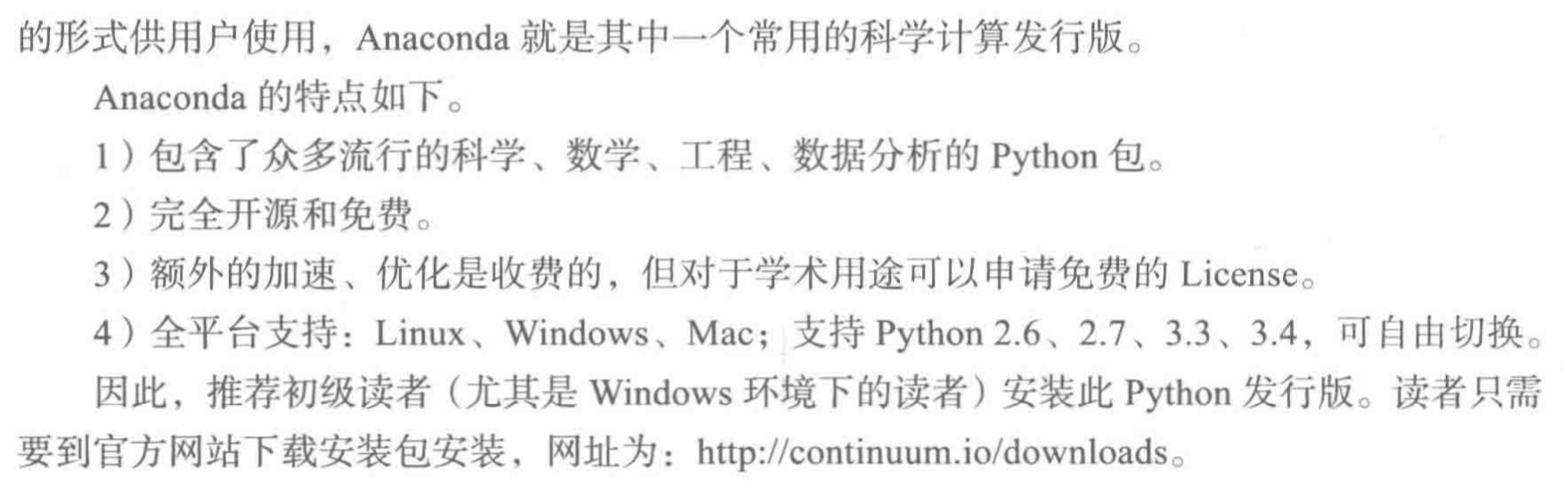Python使用入门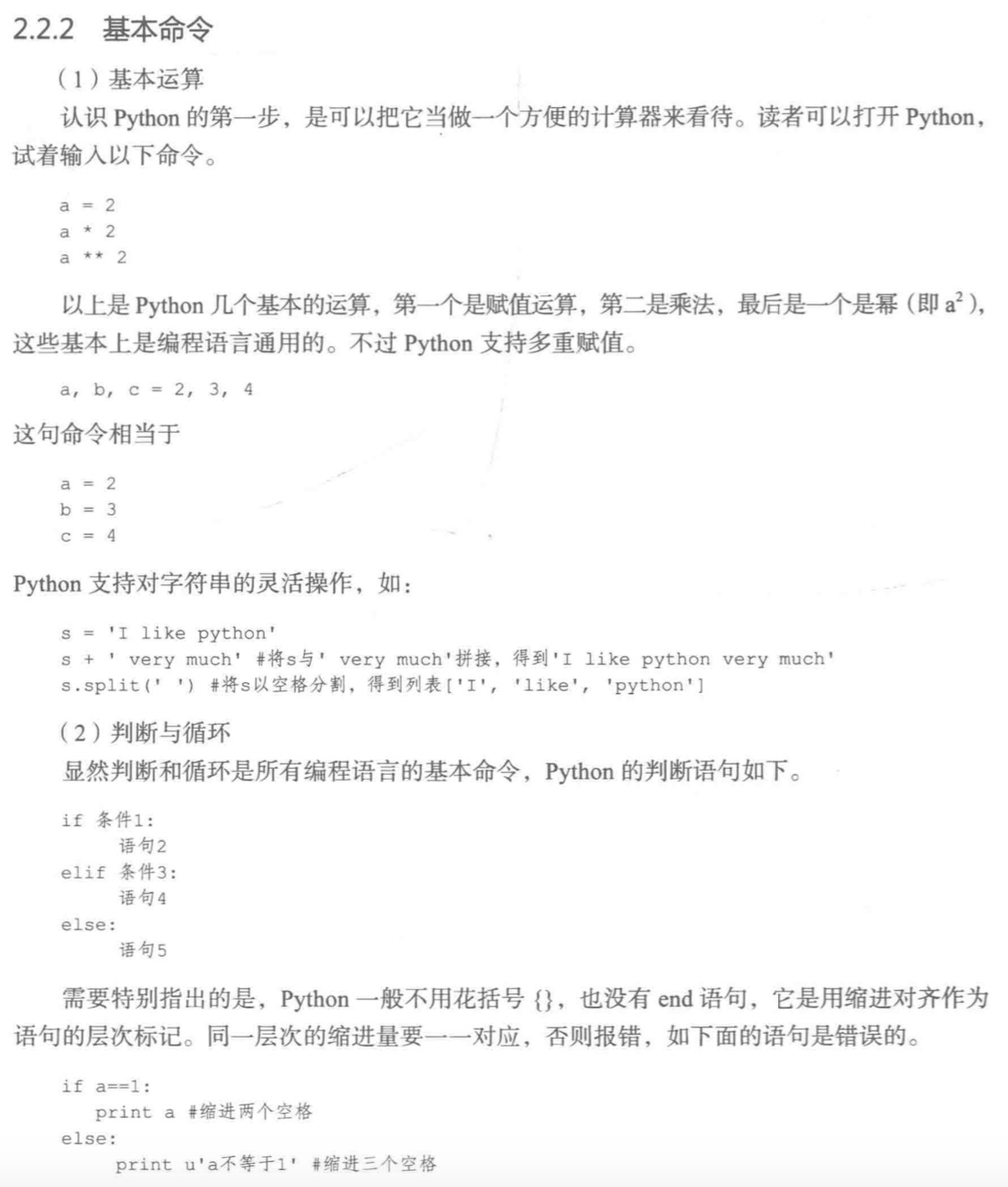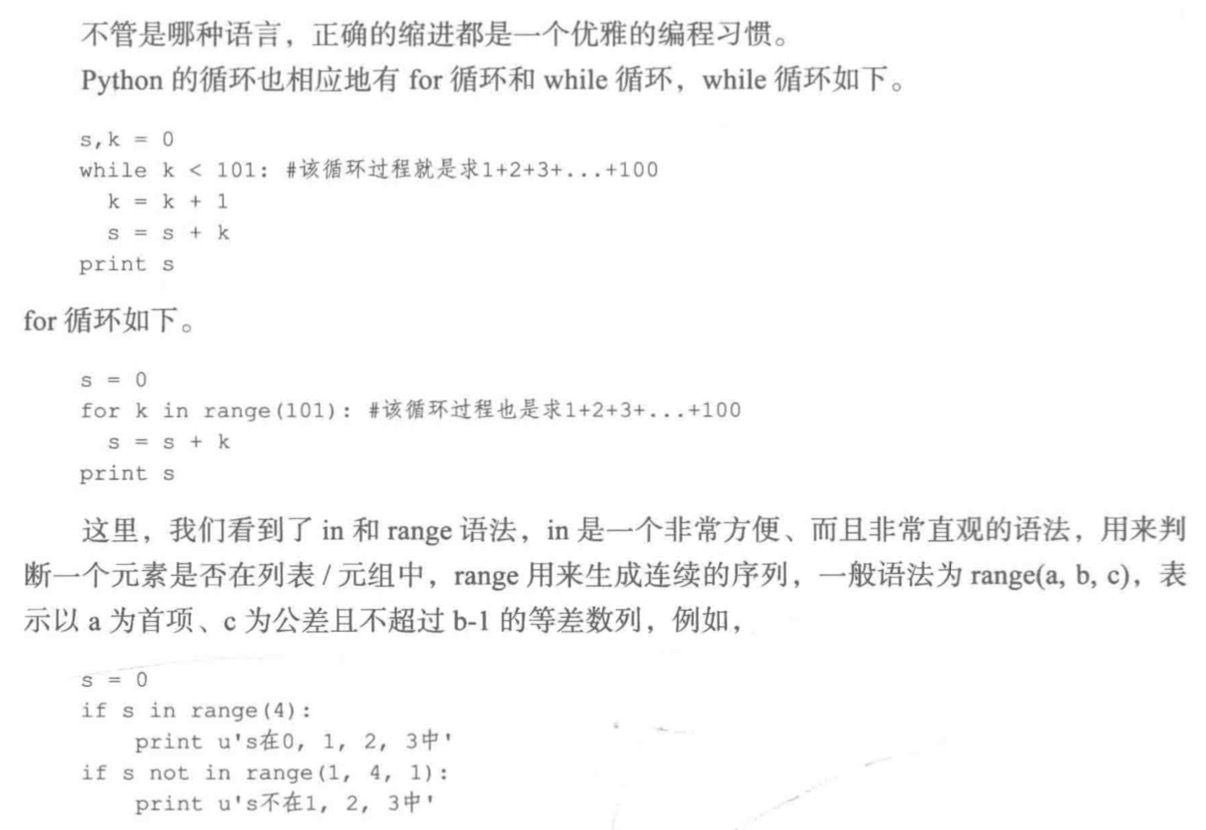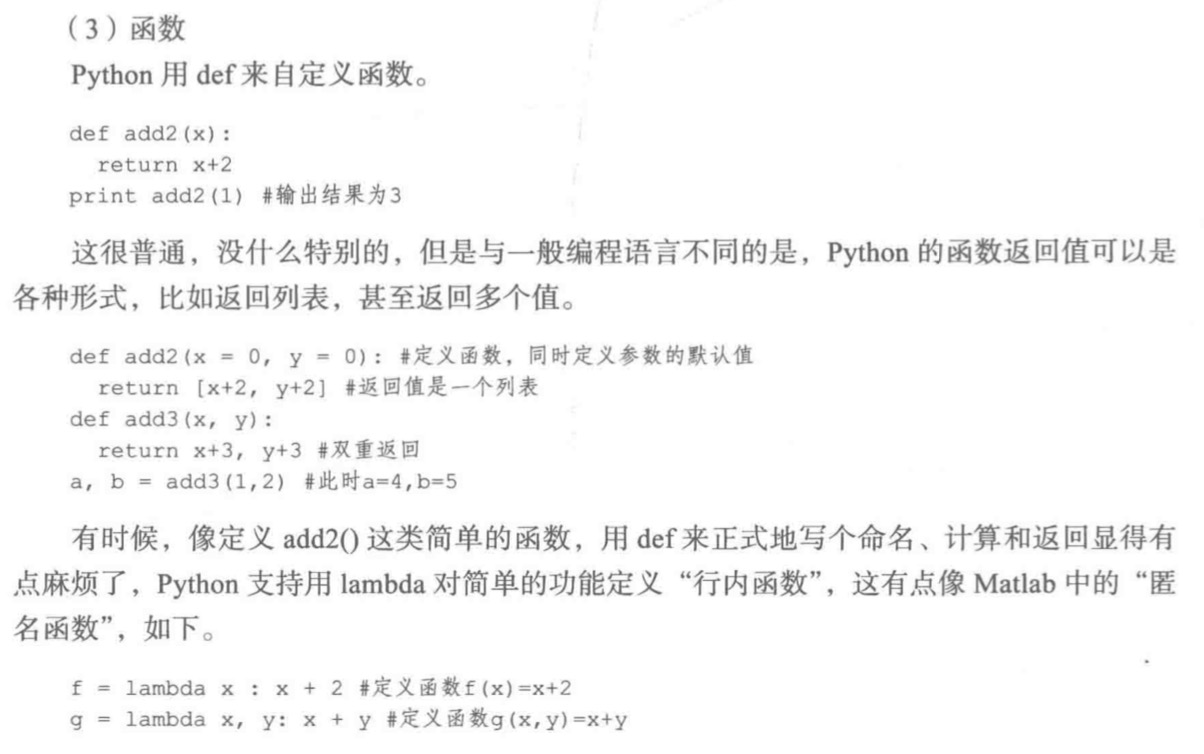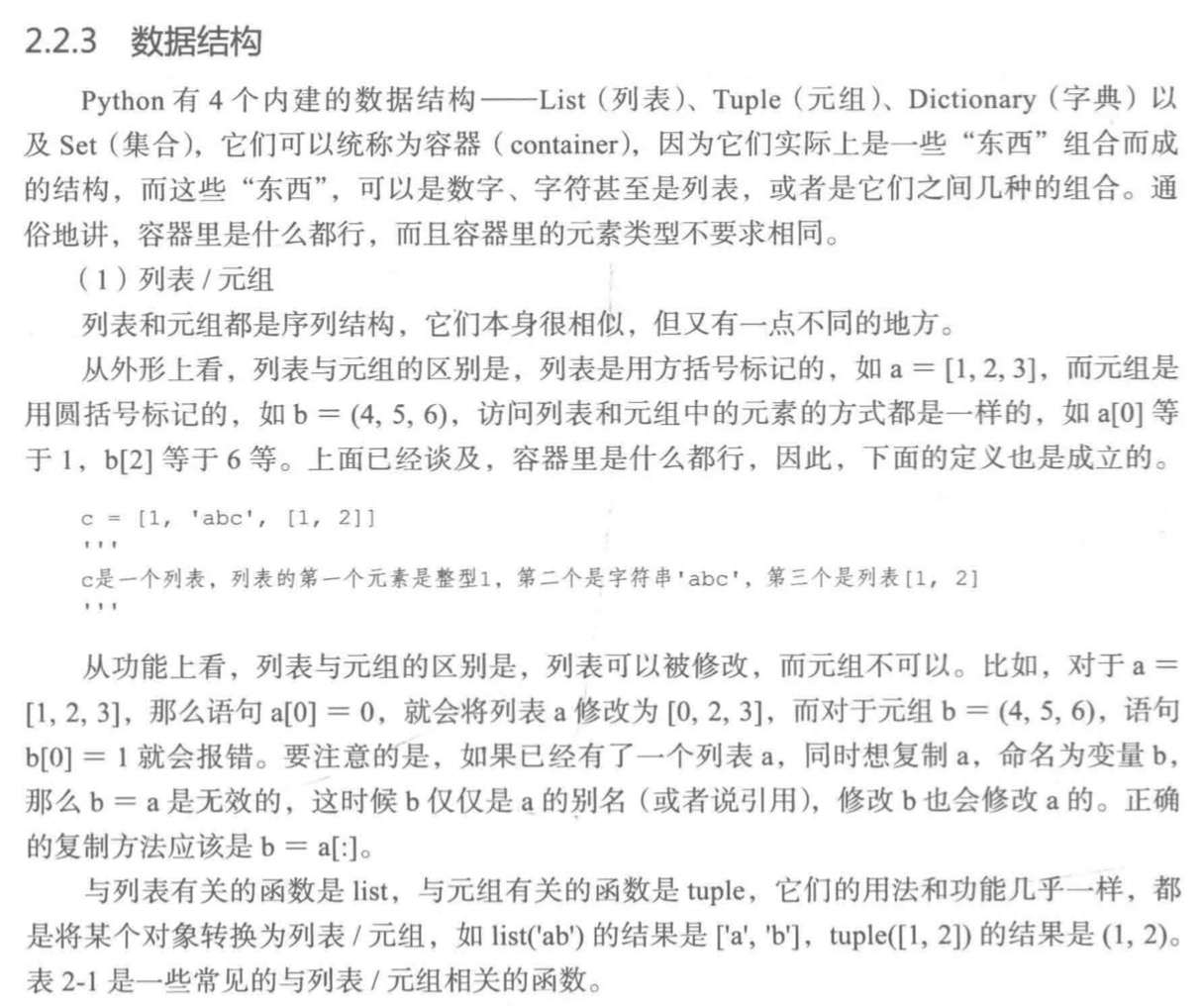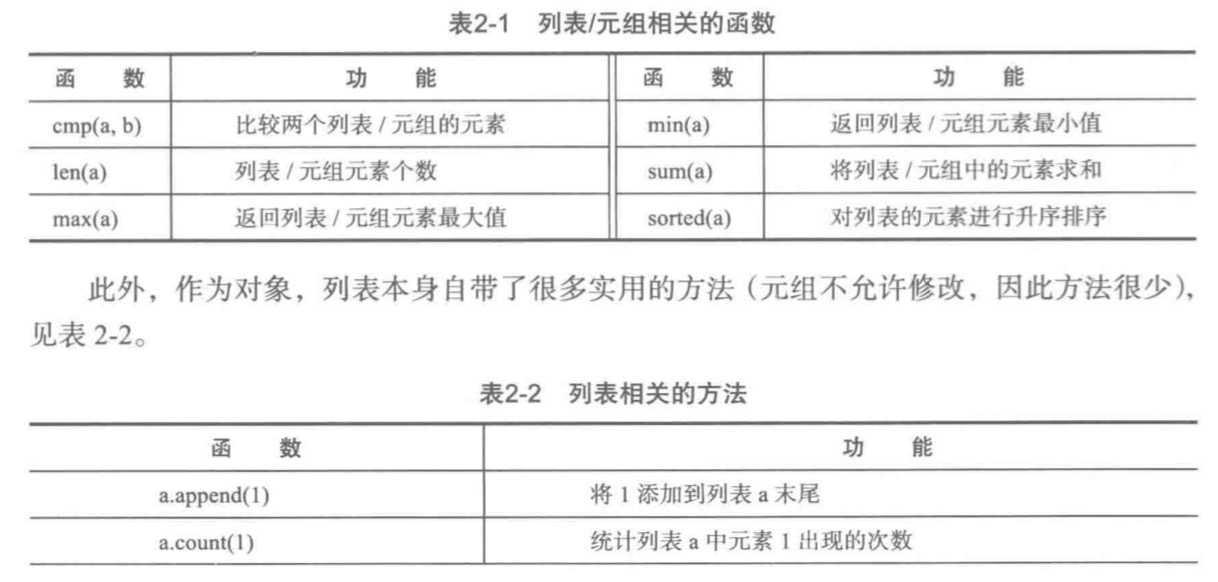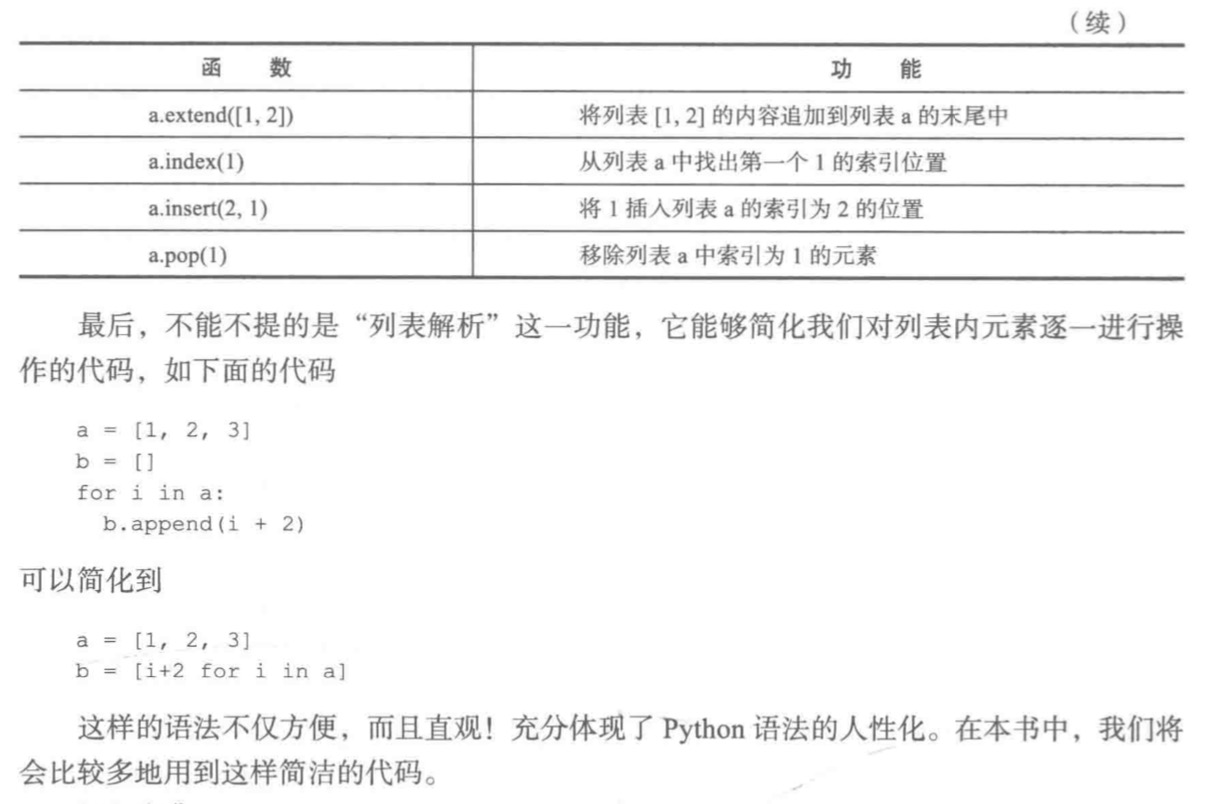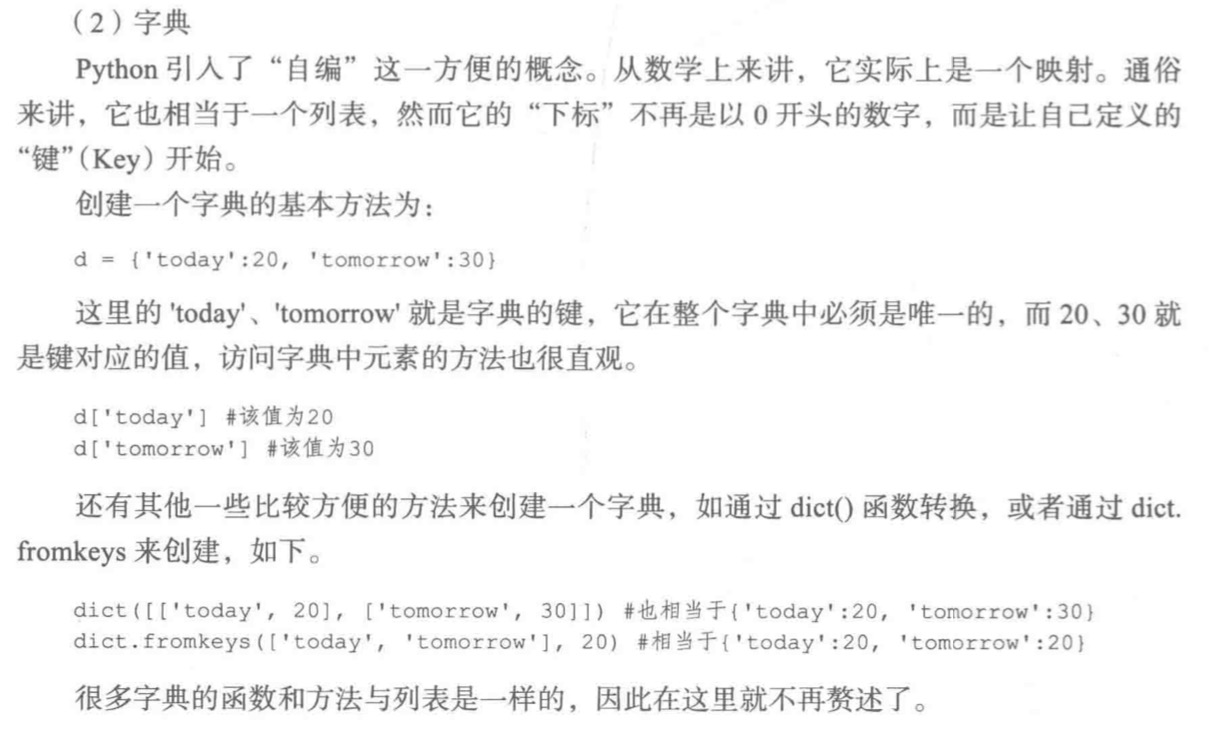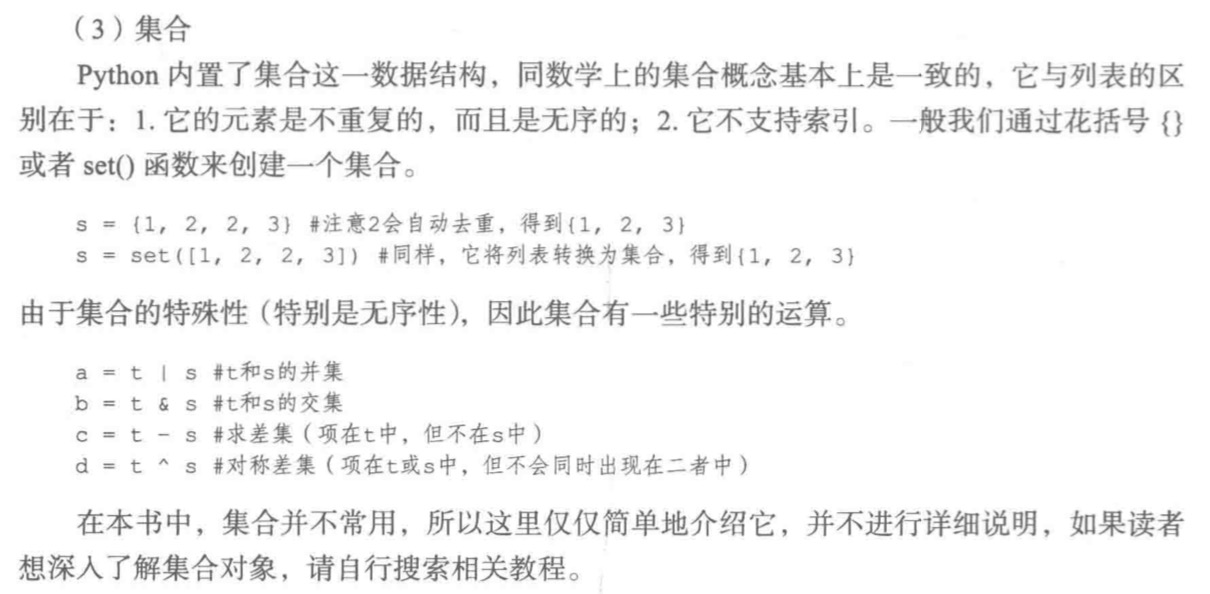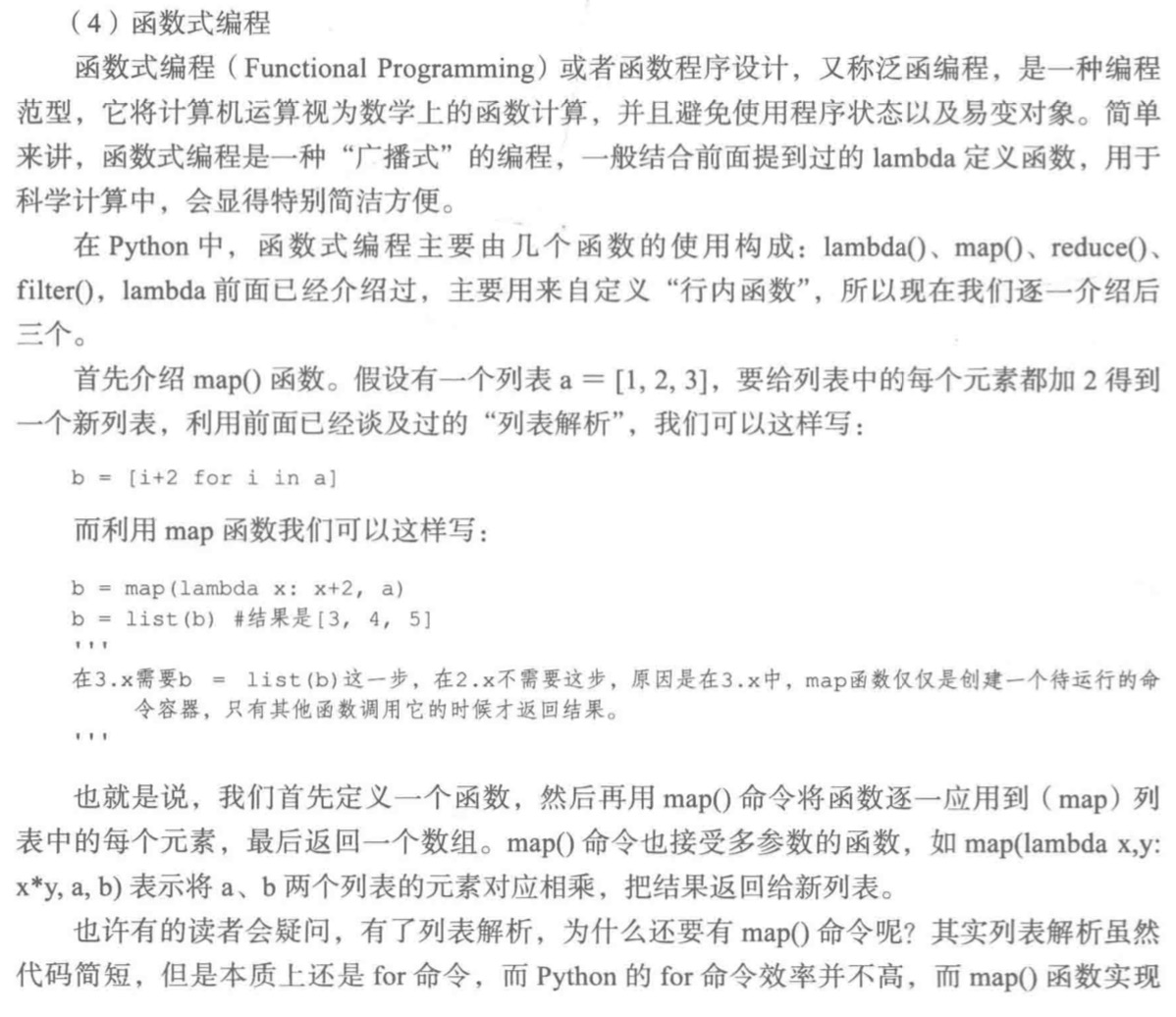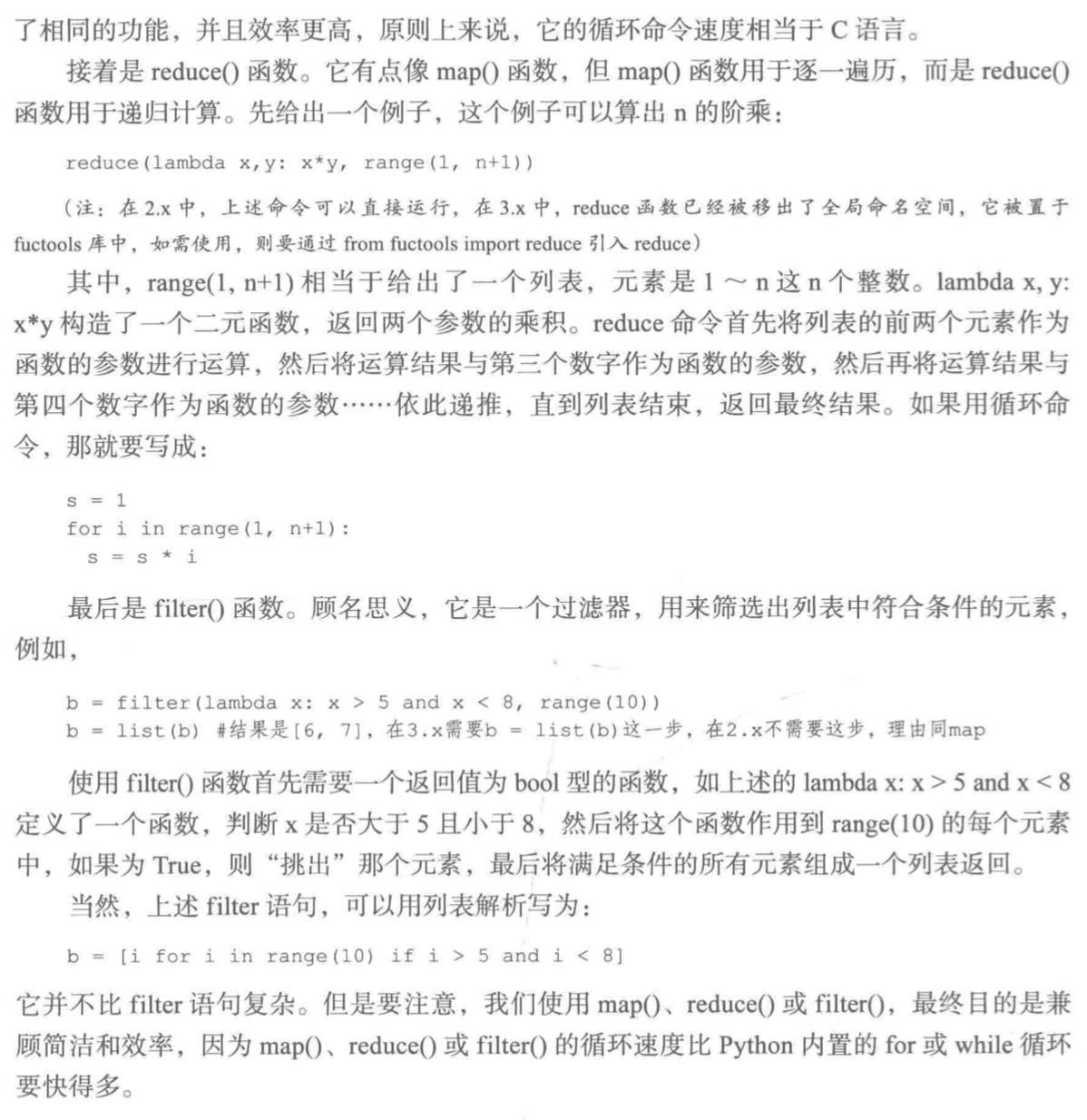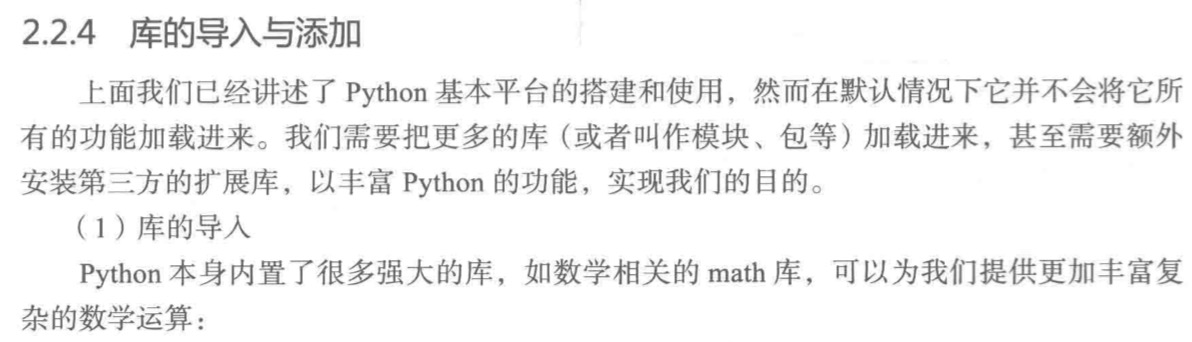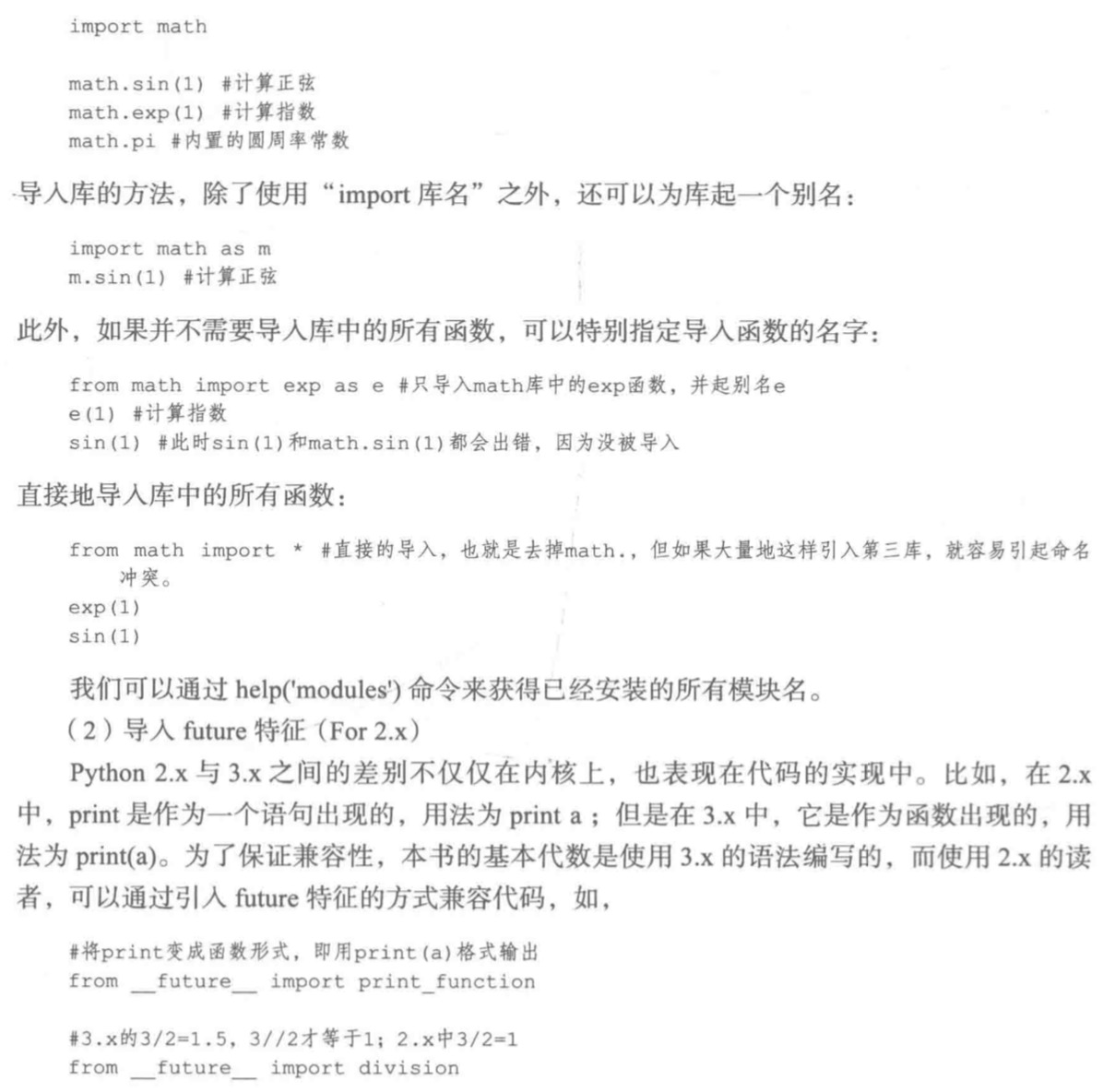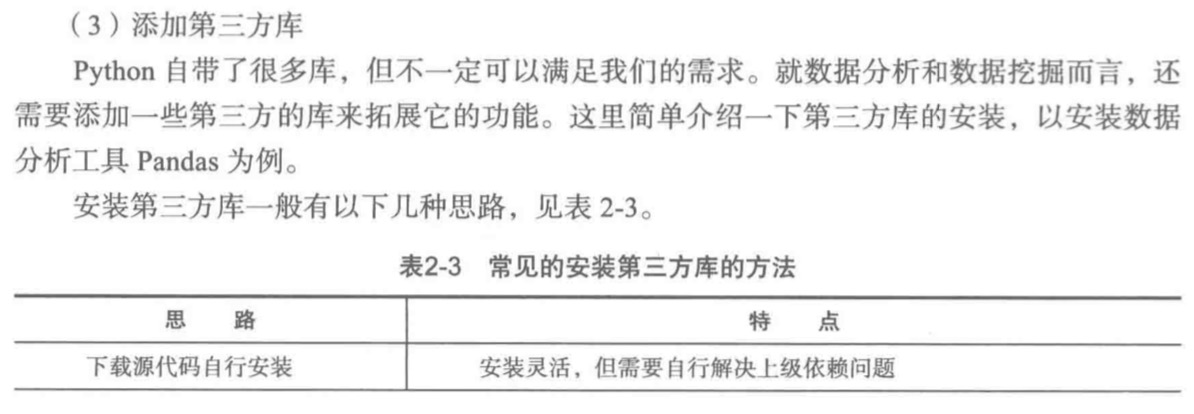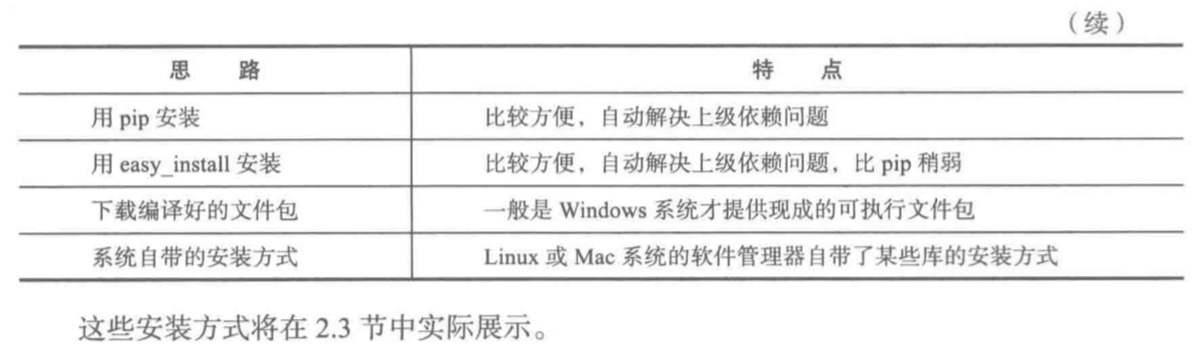Python数据分析工具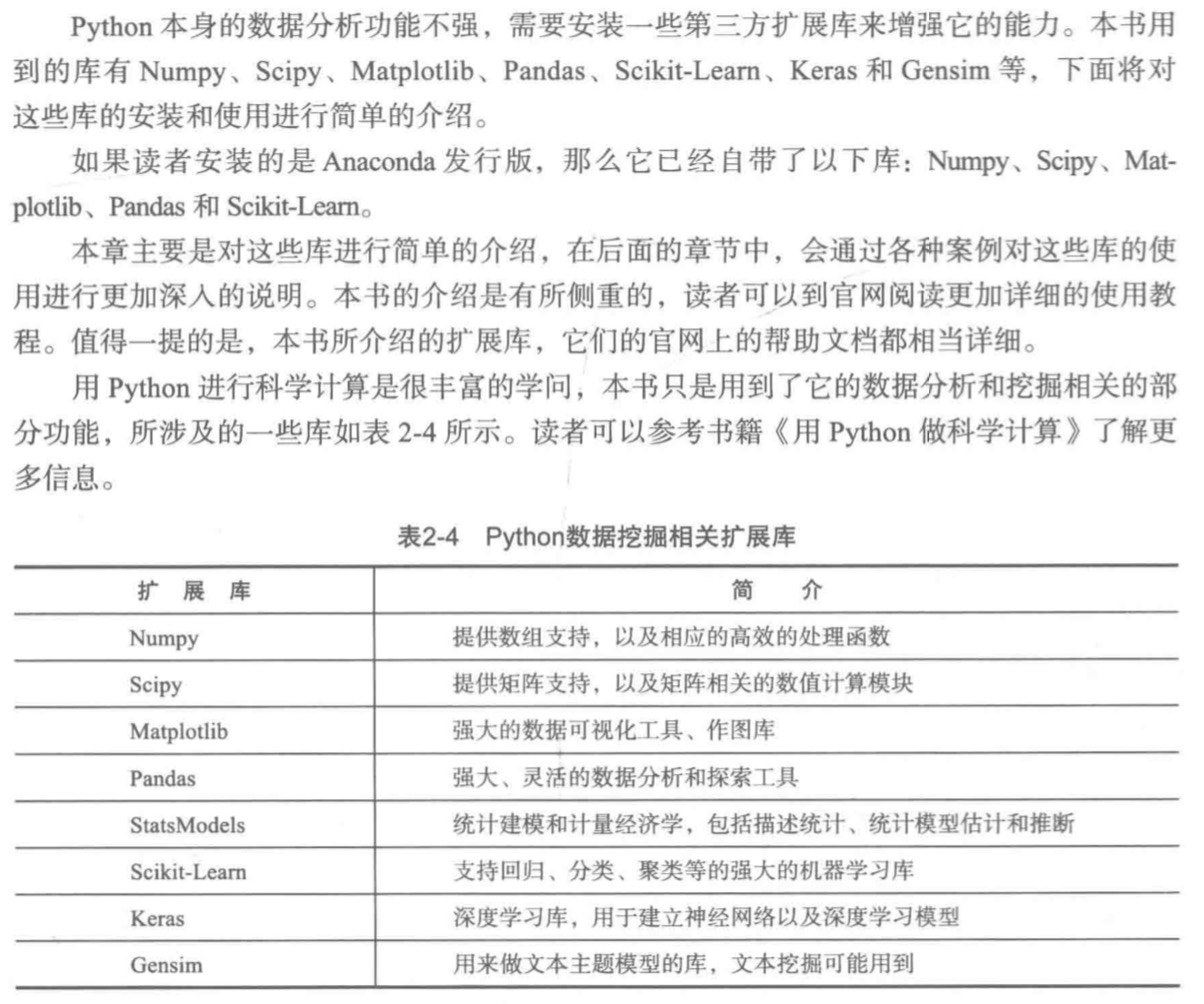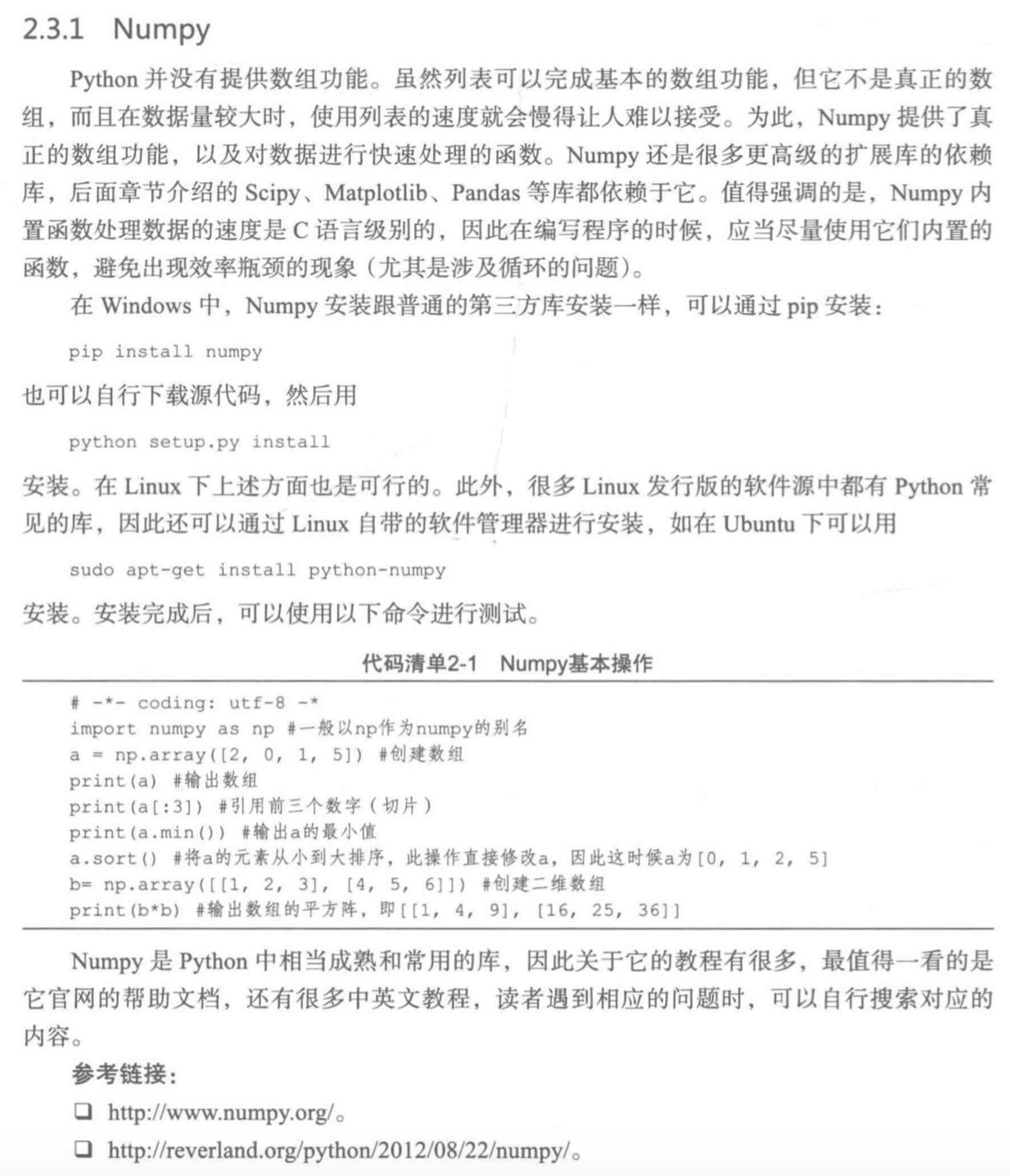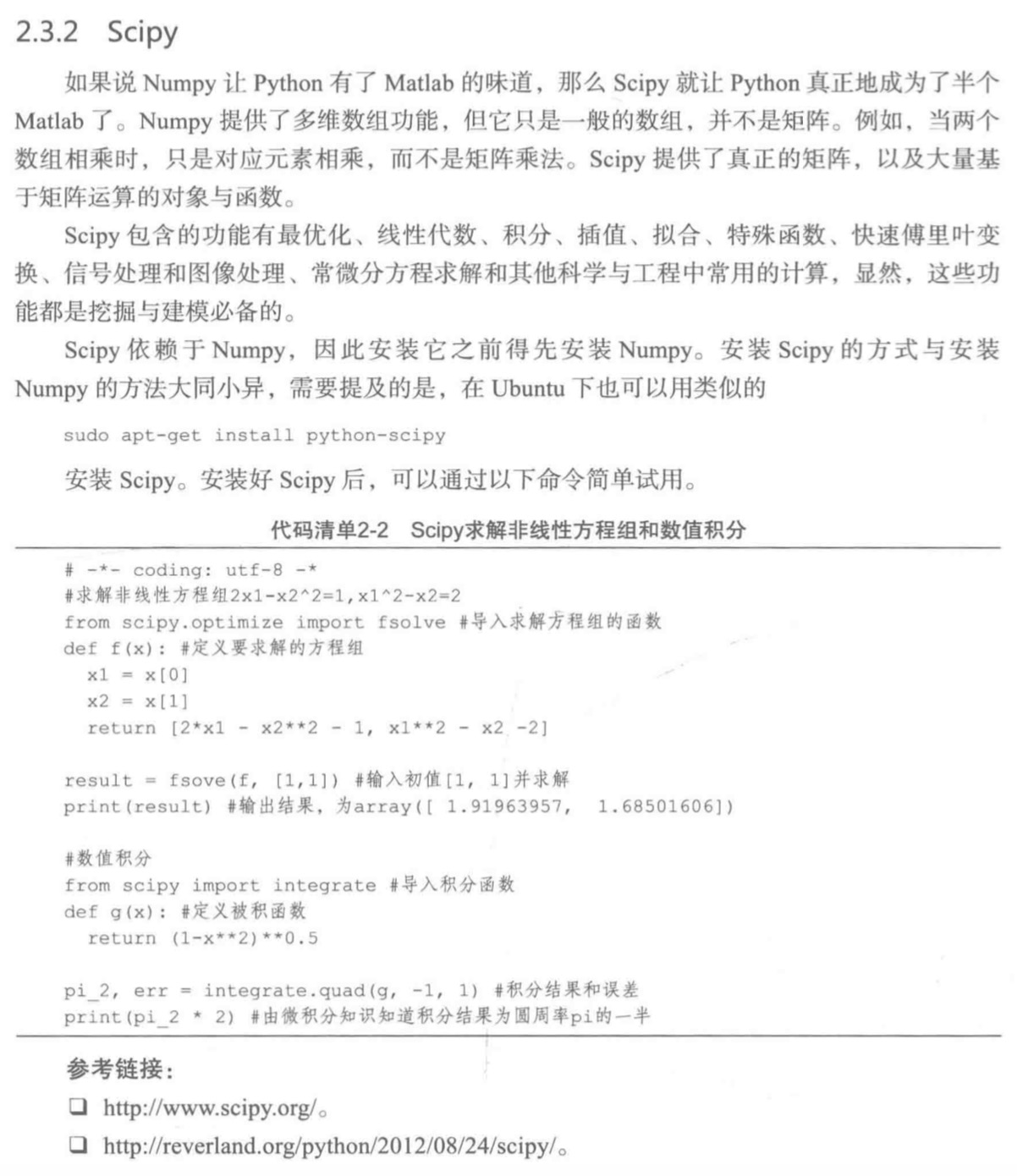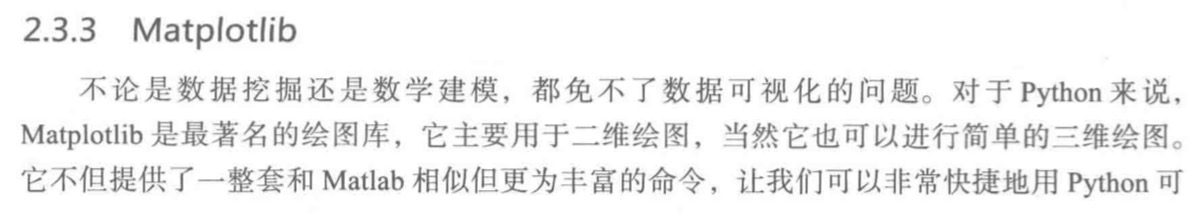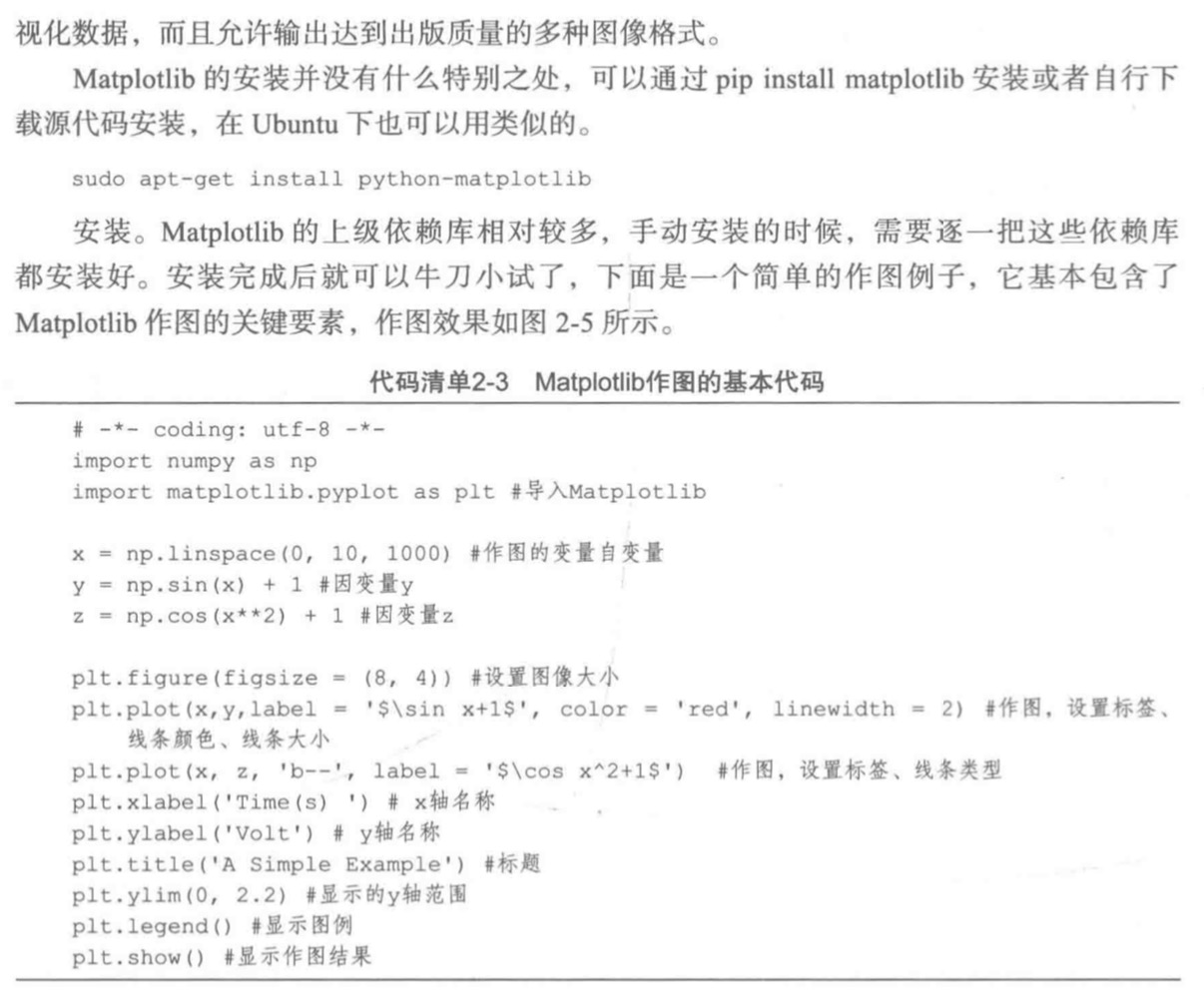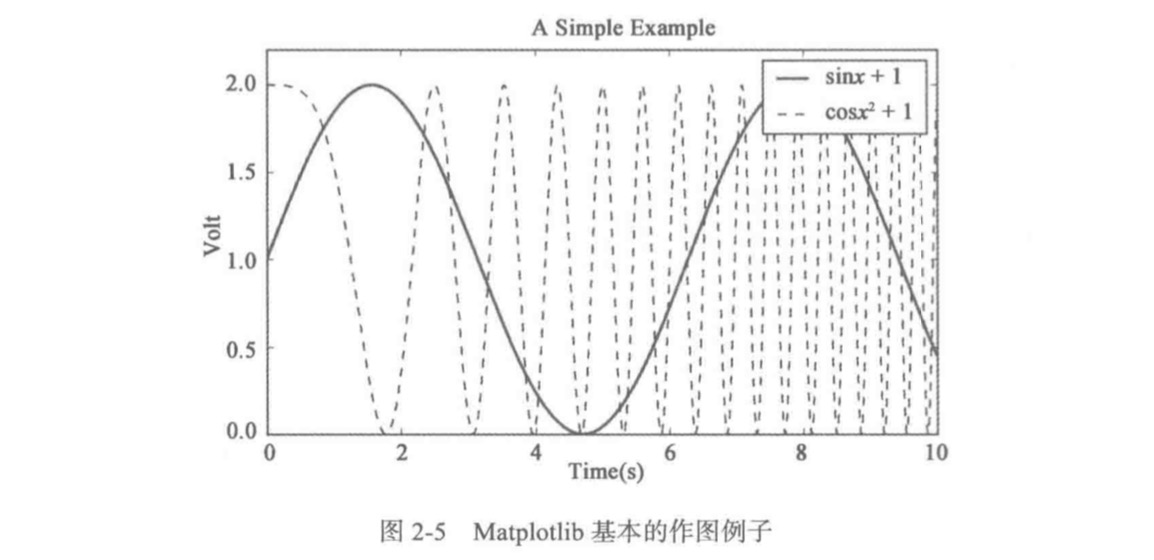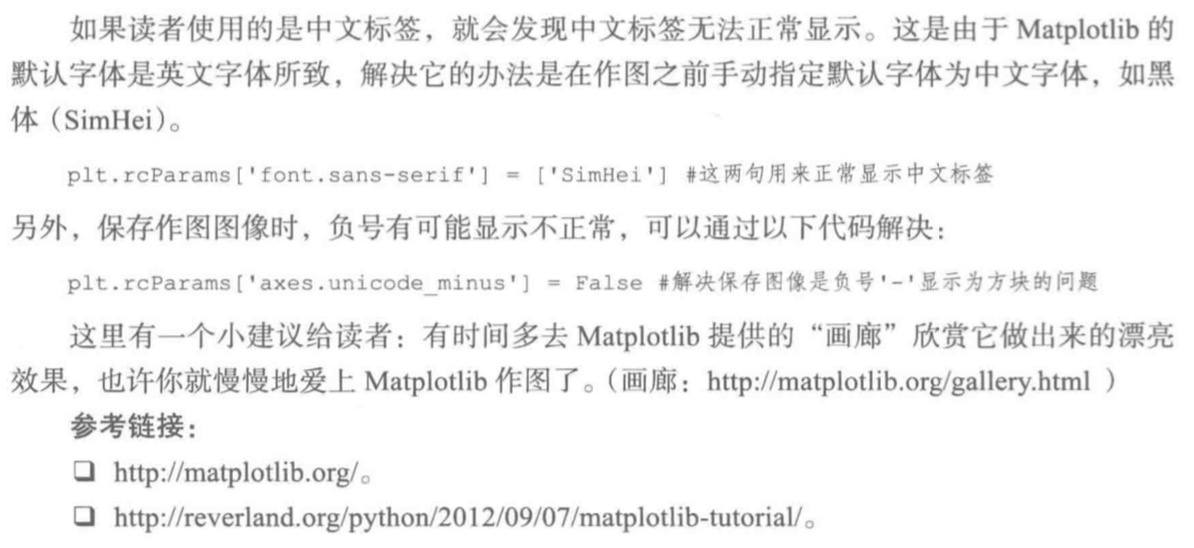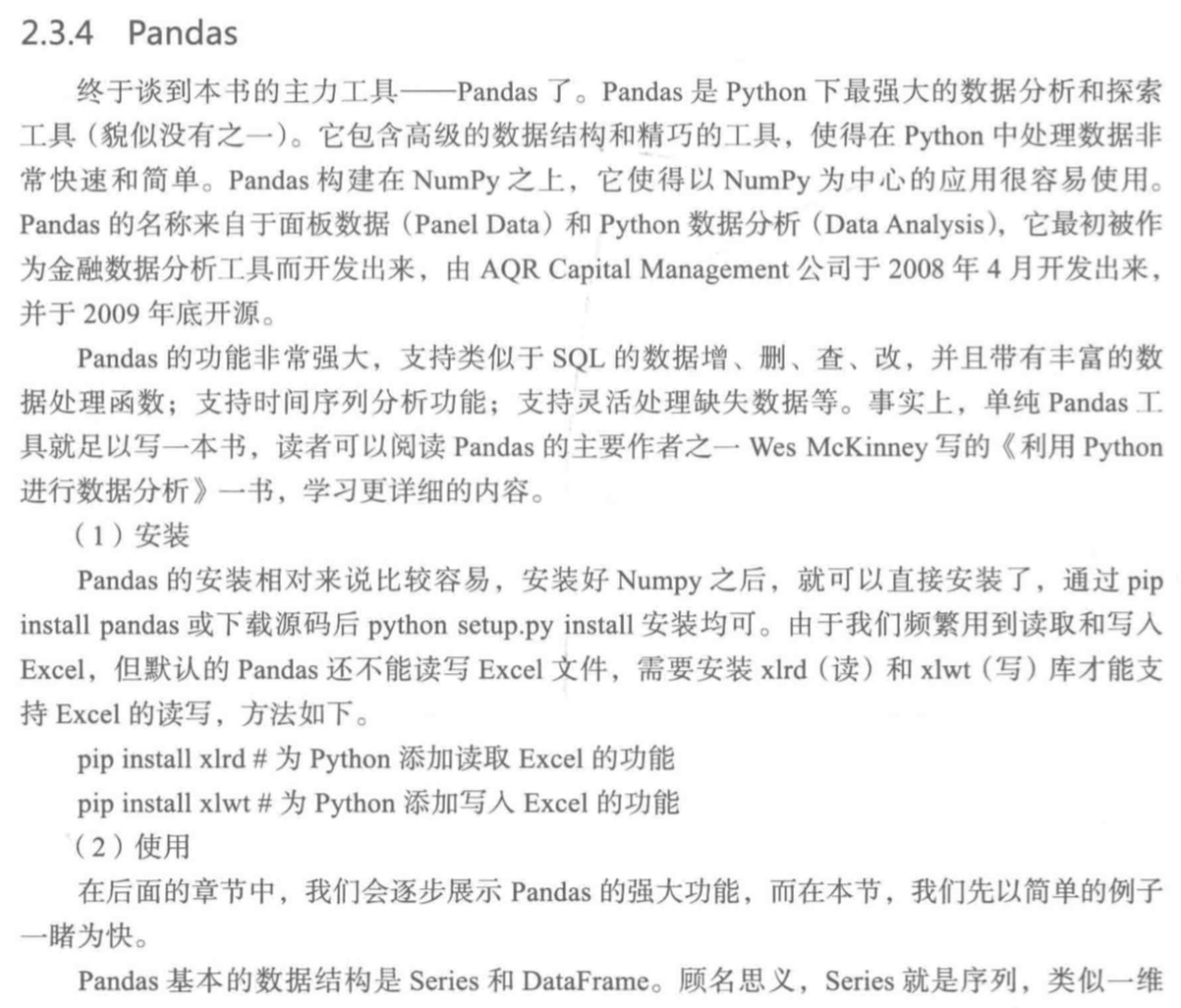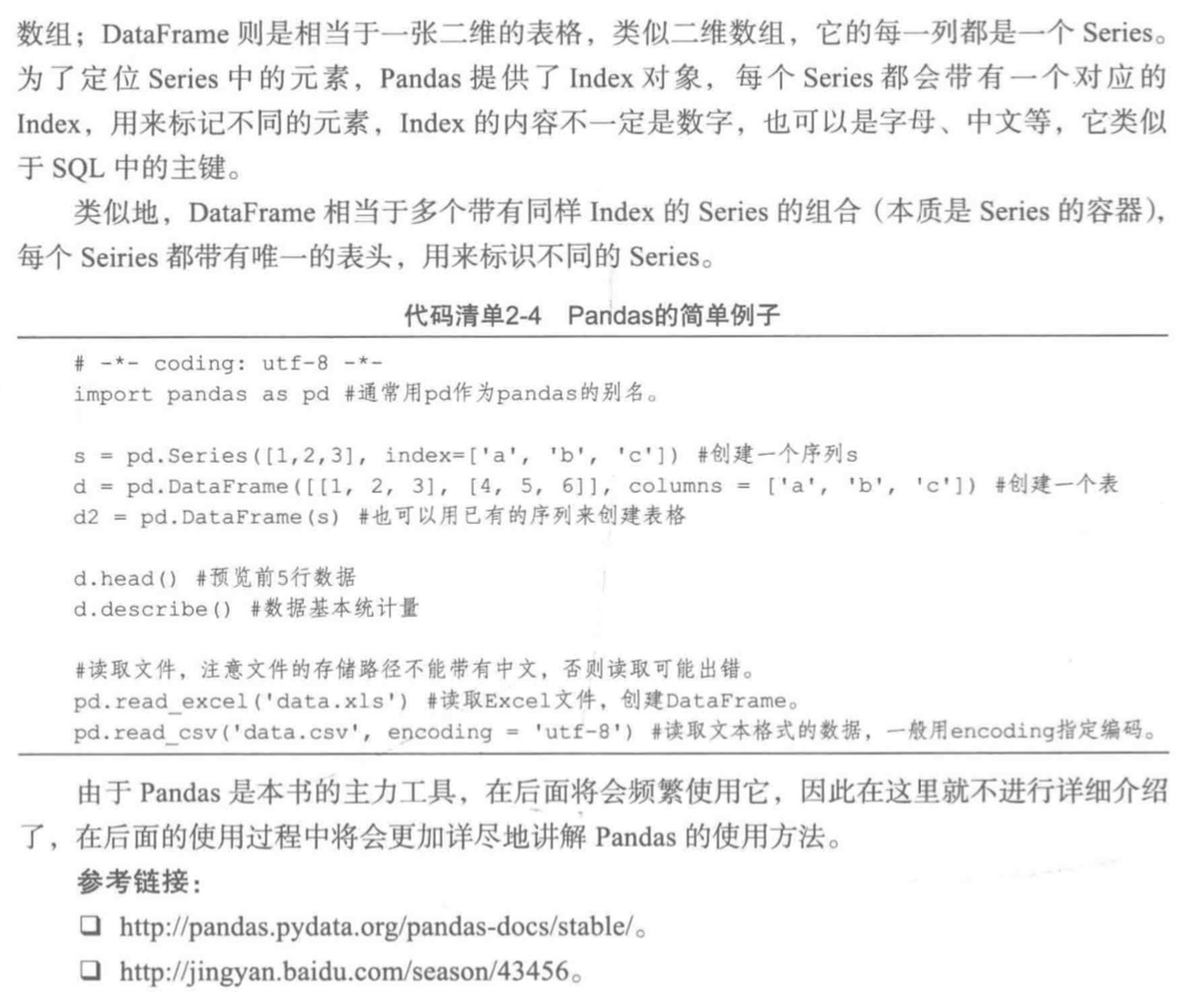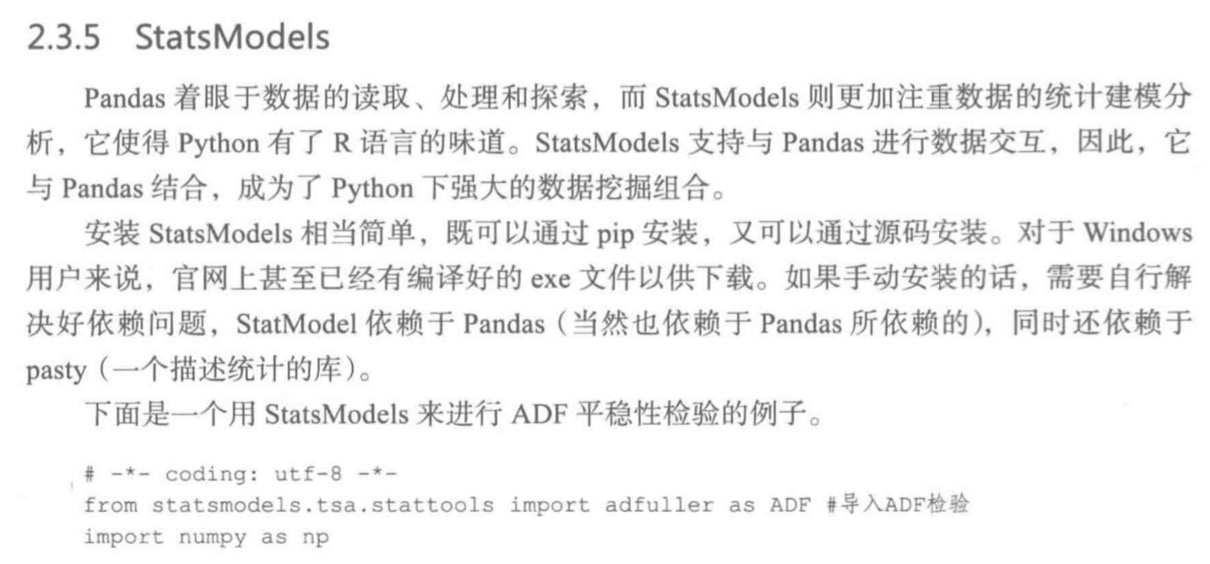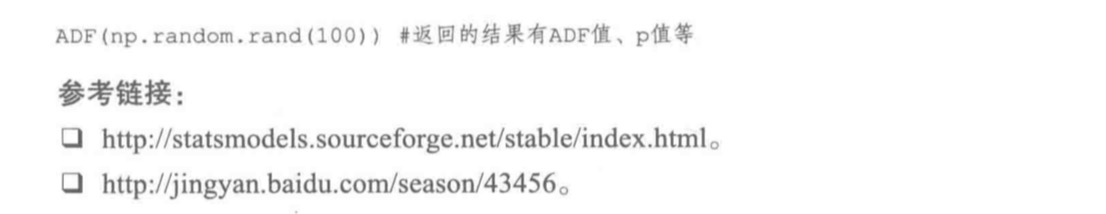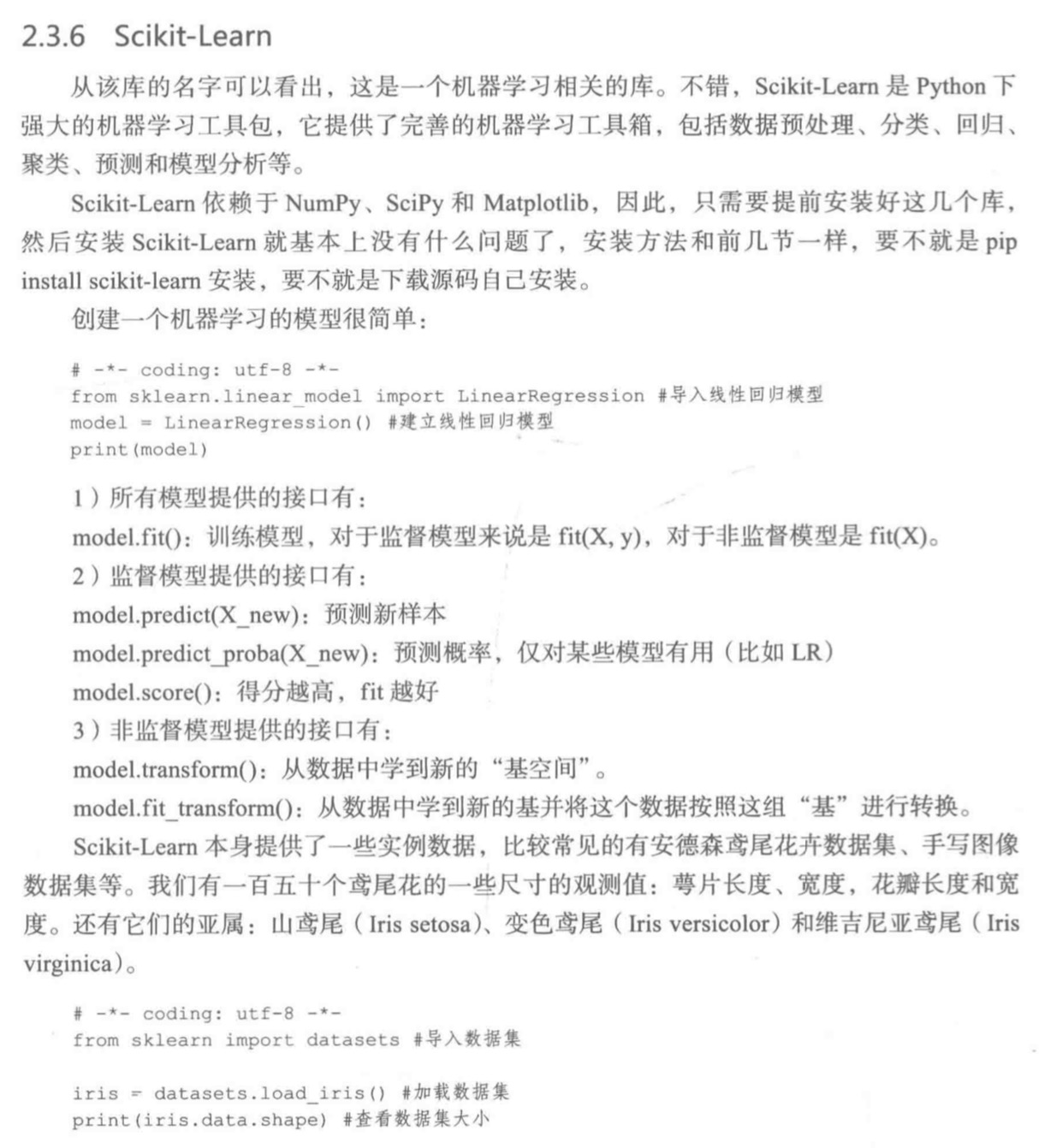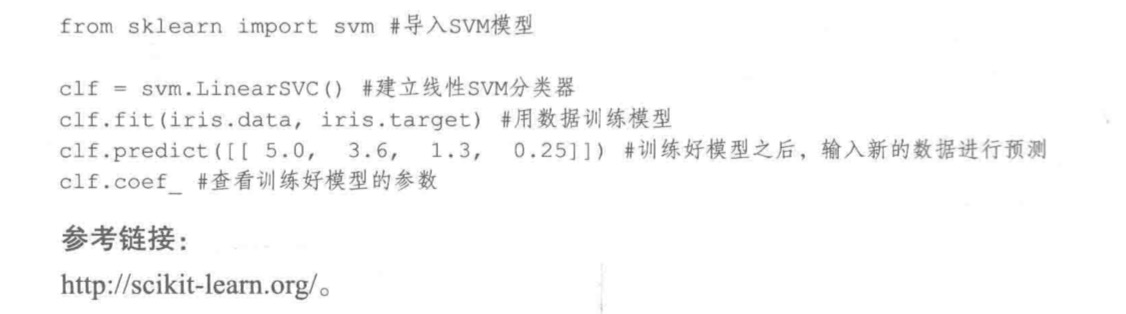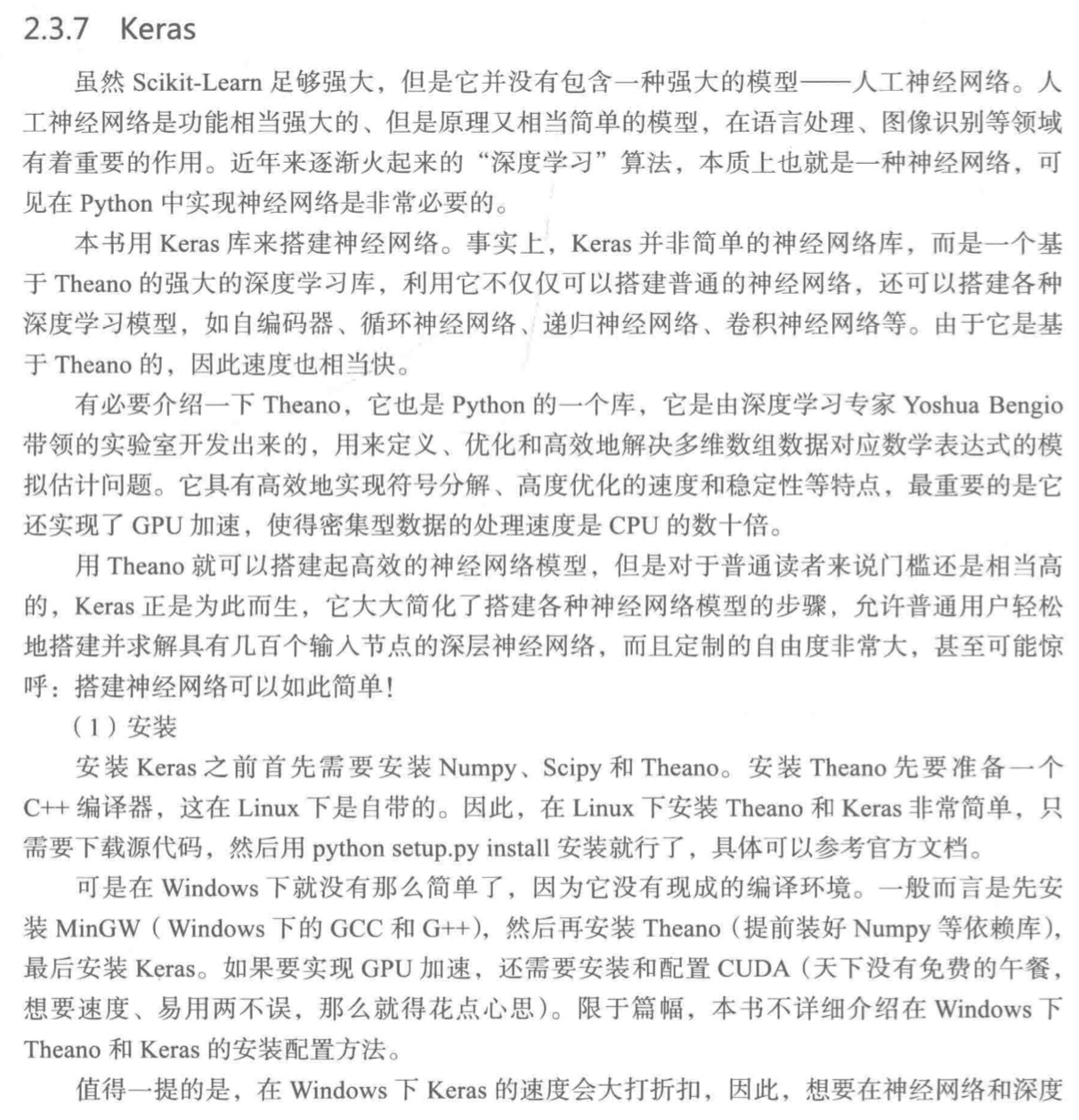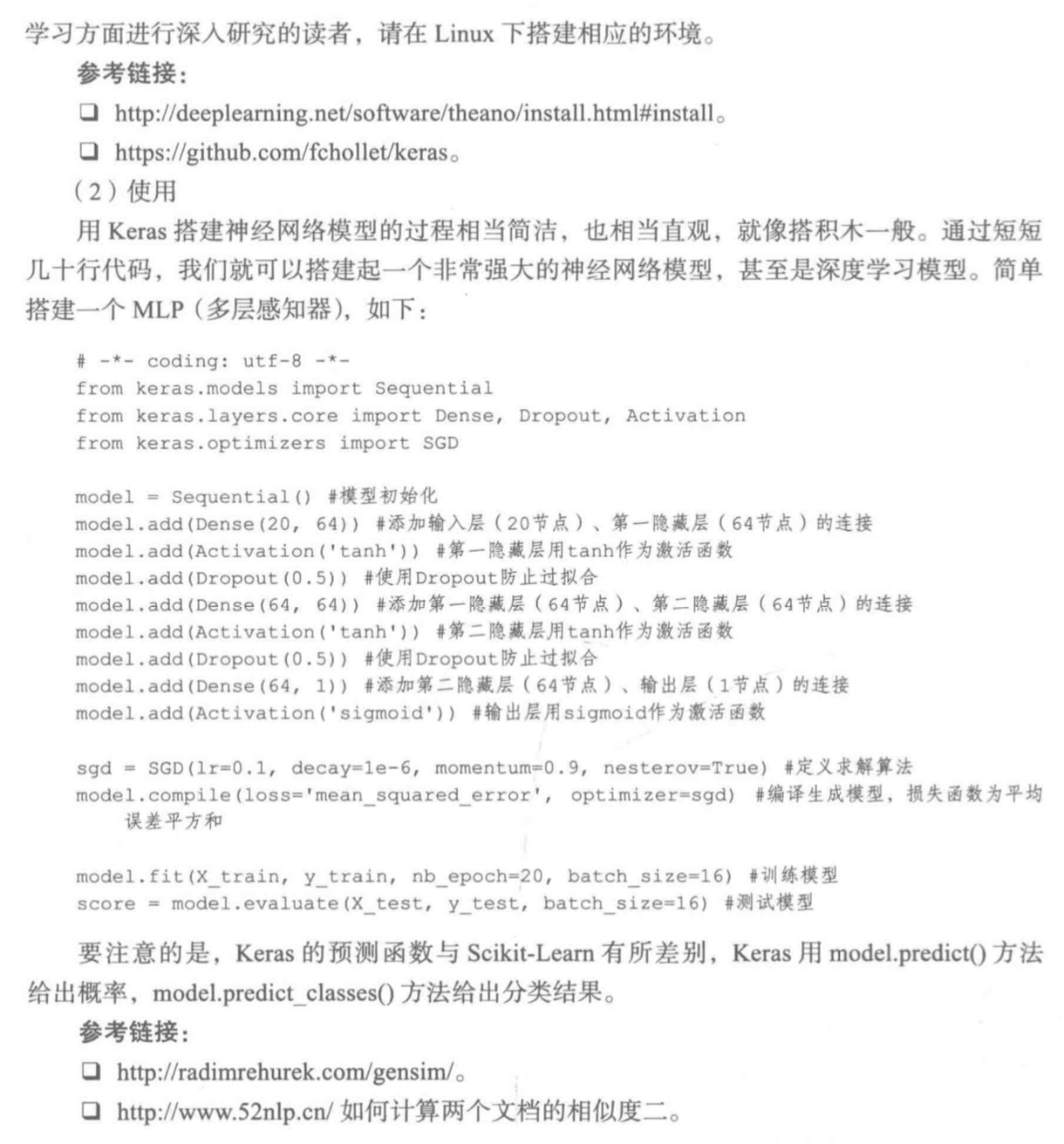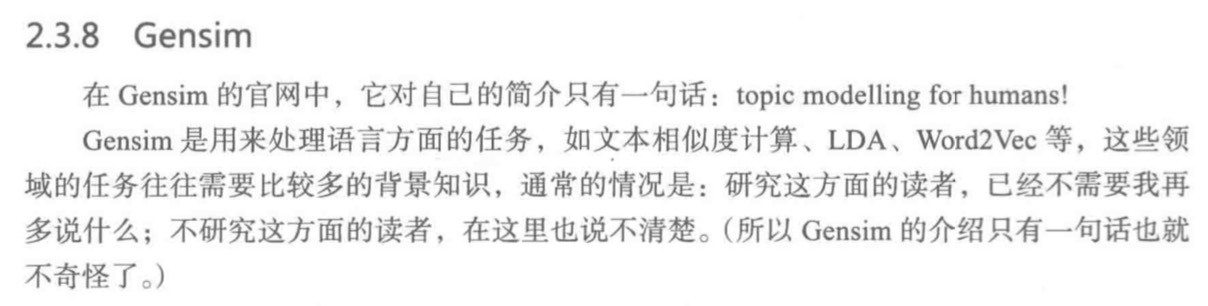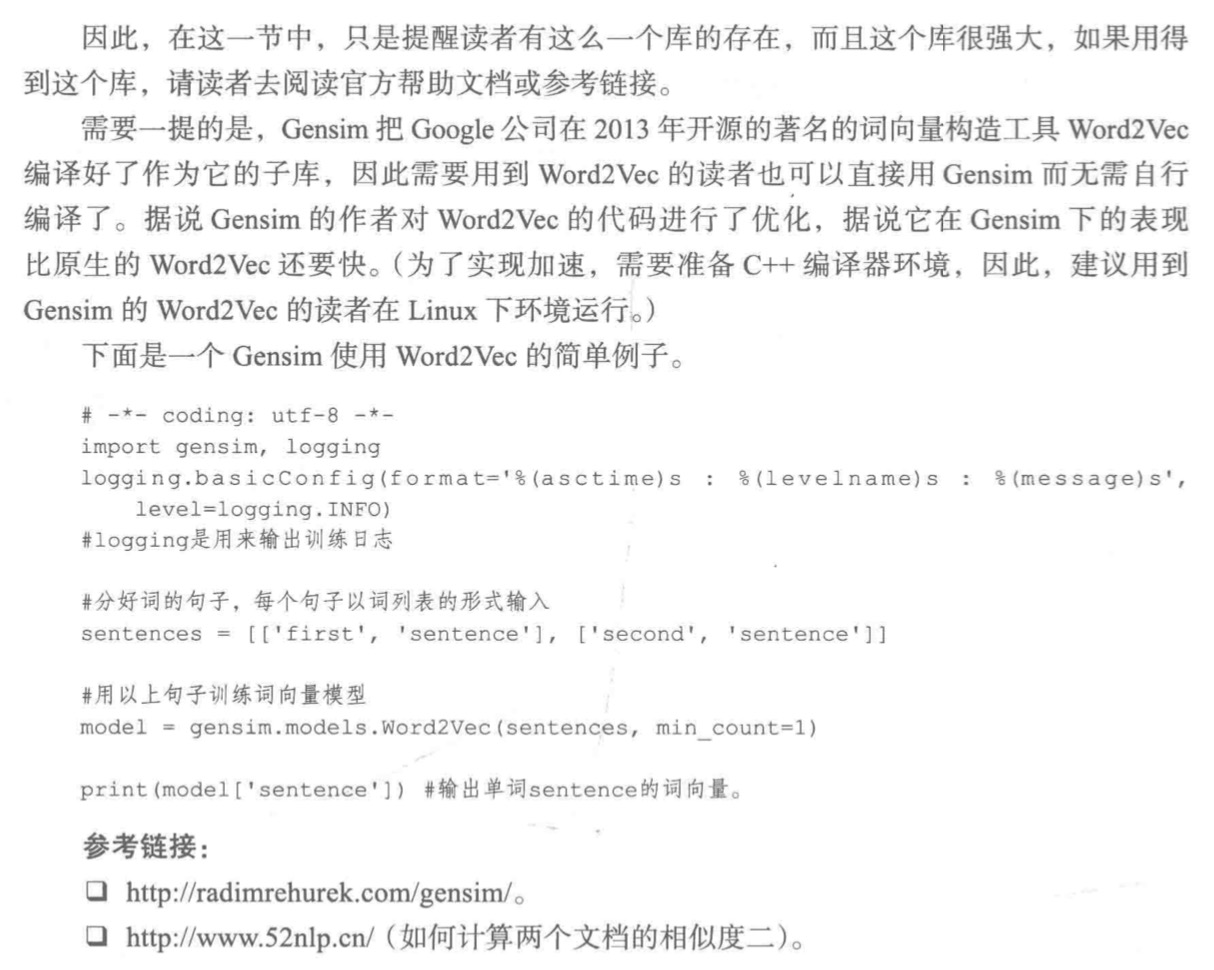配套资源使用设置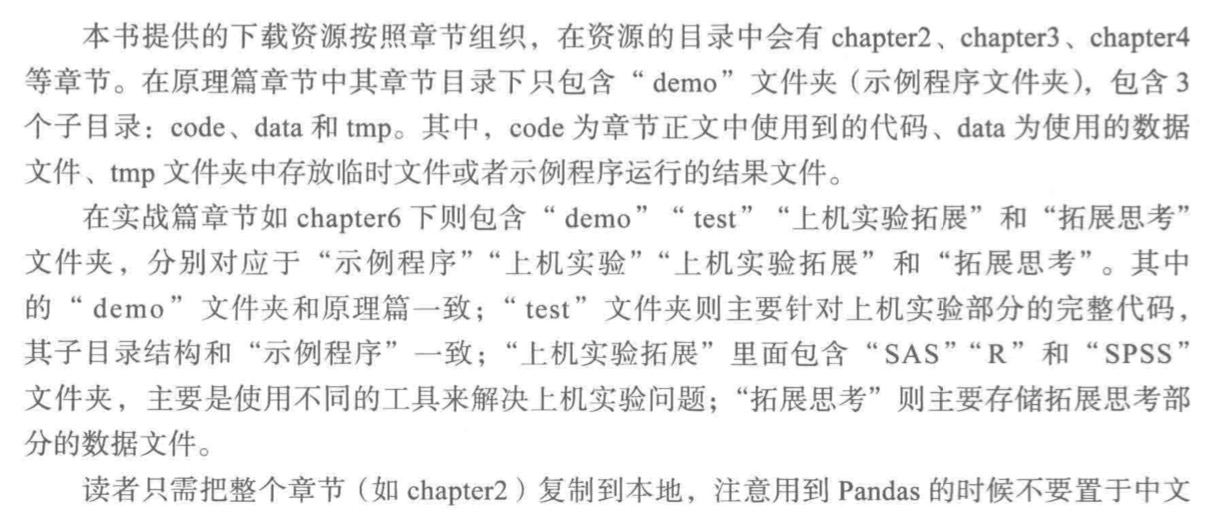展开全文• python数据分析与挖掘实战》-张良均，第五章总结的读书笔记 记录我的学习之旅，每份文档倾心倾力，带我成我大牛，回头观望满脸笑意，望大家多多给予意见，有问题或错误，请联系 我将及时改正；借鉴文章标明出处，...
• python数据分析与挖掘实战》-张良均，第三章总结的读书笔记 记录我的学习之旅，每份文档倾心倾力，带我成我大牛，回头观望满脸笑意，望大家多多给予意见，有问题或错误，请联系 我将及时改正；借鉴文章标明出处，...
• python数据分析与挖掘实战 pdf 张良均 资源共享 网盘下载： https://pan.baidu.com/s/1jQuthI-7NSDO5GWylu7YkQ 无提取码 永久有效
• Python数据分析与挖掘实战-----第1章 数据挖掘基础Python数据分析与挖掘实战-----第1章 数据挖掘基础数据挖掘的基本任务数据挖掘建模过程定义挖掘目标数据取样数据探索数据预处理挖掘建模模型评估常用数据挖掘建模...
• 文章目录欢迎购买正版书籍 欢迎购买正版书籍 豆瓣评价：Python数据分析与挖掘实战 作者: 张良均 / 王路 / 谭立云 / 苏剑林 出版社: 机械工业出版社 参考源码：《python数据分析与挖掘实战》的代码笔记 ...学习笔记 数据探索
• 豆瓣评价：Python数据分析与挖掘实战 作者: 张良均 / 王路 / 谭立云 / 苏剑林 出版社: 机械工业出版社 参考源码：《python数据分析与挖掘实战》的代码笔记 第一章数据挖掘基础 1.数据挖掘的基本...学习笔记 基础
• python数据分析与挖掘实战学习笔记（一）数据挖掘所用的python工具Numpy的下载安装Scipy的下载安装Matplotlib的下载安装Pandas的下载安装Scikit-learn的下载安装Keras的下载安装 数据挖掘所用的python工具 Numpy,...
• Python数据分析与挖掘实战-----第5章 挖掘建模Python数据分析与挖掘实战-----第5章 挖掘建模分类预测聚类分析关联规则时序模式离群点检测 Python数据分析与挖掘实战-----第5章 挖掘建模 分类预测 聚类分析 关联...
• Python数据分析与挖掘实战-----第3章 数据探索Python数据分析与挖掘实战-----第3章 数据探索数据质量分析数据特征分析Python主要数据探索函数 Python数据分析与挖掘实战-----第3章 数据探索 数据质量分析 ...
• Python数据分析与挖掘实战-----第4章 数据预处理Python数据分析与挖掘实战-----第4章 数据预处理数据清洗数据集成数据变换数据规约Python主要数据预处理函数 Python数据分析与挖掘实战-----第4章 数据预处理 数据...
• 最近在学习张良均老师的《Python数据分析与挖掘实战》，发现部分代码存在错误，特分享调试好的代码，供学习参考。 代码清单4-1 用拉格朗日法进行插补 书本代码： #拉格朗日插值代码 import pandas as pd #导入...
• 本文是基于《Python数据分析与挖掘实战》的实战部分的第15章的数据——《电商产品评论数据情感分析》做的分析。旨在回顾对评论文本数据的处理和建模方法。1 挖掘背景目标 对京东平台上的热水器评论进行文本挖掘...文本分析
• 一个利用机器学习做数据挖掘实战的小项目，参考书为《Python数据分析与挖掘实战》。 项目诞生的原因 本身自己就算菜鸟，熟悉github也算目的 《Python数据分析与挖掘实战》是一本很适合有一定的机器学习理论的人尝试...
• 文章目录欢迎购买正版书籍 欢迎购买正版书籍 豆瓣评价：Python数据分析与挖掘实战 作者: 张良均 / 王路 / 谭立云 / 苏剑林 出版社: 机械工业出版社 参考源码：《python数据分析与挖掘实战》的代码笔记 ...学习笔记
• Python数据分析与挖掘实战 代码清单3-3更正 代码清单3-3 菜品盈利帕累托图代码 书上代码如下from __future__ import print_functionimport pandas as pd#初始化参数dish_profit = '../data/catering_dish_profit....
• python数据分析与挖掘实战第五章挖掘建模例5-3神经网络算法预测销量高低神经网络散发预测销量高低#-*- coding: utf-8 -*-#使用神经网络算法预测销量高低import pandas as pd#参数初始化inputfile = '../data05/sales...代码纠错 神经网络算法预测
• 本文是《Python数据分析与挖掘实战》的实战部分的第10章的数据——《家用电器用户行为分析事件识别》做的分析。 1.背景挖掘目标 国内某智能热水器，在状态发生改变或有水流状态时会采集数据，该厂商根据采集到的......

# python数据分析与挖掘实战python 订阅